﻿ correct Bohr model of Helium

# Quantum mechanics is wrong.

Youhei Tsubono, Japan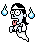Criticize the present physics.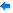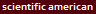(20/ 9/18 )

## Quantum mechanics is nonsense.

### [ A single electron can pass both slits at the same time !? ]

(Fig.1) Quantum mechanics = Many worlds = Fantasy.The present physics is filled with unreal and paradoxical concepts such as parallel worlds even in academic organization.

Why did we fall into such a miserable situation ?

In Quantum mechanics, Schrodinger wavefuntion is useless, it only gives vague probability density of each electron.

This dubious wavefunction is the origin of strange ideas such as many-worlds where an electron can be in all possible states at the same time.

And it misleads people and entangle them in an endless debate about "consciousness", which is philosophy rather than science.

## The theory of everything = extra-dimensions !

### [ 10-dimensional string theory is the only unified theory. ]

(Fig.2)  Quantum mechanics + Einstein relativity = string theory.Theory of everything is an unified theory of quantum mechanics and Einstein's general relativity.

The only theory of everything accepted now is string ( M ) theory, which relies on unreal extra-dimensions.

They believe quixotic idea that our universe is made of 10 ( or 11 ) dimensional spacetime instead of 4 (= x,y,z + time ) !

Unfortunately, this fanciful string theory is the only mainstream unified theory, so it monopolizes all important academic positions.

It means unless you believe this string theory, you'll be surelly kicked out of academy and cannot be professors, let alone famous.

Surprisingly, this only theory of everything depends on wrong math ( 1 + 2 + 3 = ∞ = - 1/12 ) and 10500 different worlds, which cannot predict anything.

## The present physics believes parallel universes !

### [ Big Bang → multi-universes were born ? ]

(Fig.3)  Multiverse = Parallel universe are rampant.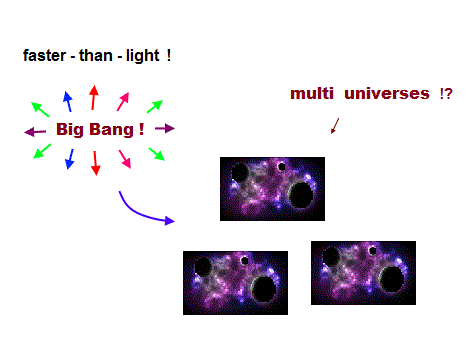Surprisingly, the present mainstream physicists believe fantasy multiverse where many universes exist parallel to each other.

Even first-rate physicists are not exception.
The present cosmology is based on fanciful faster-than-light expansion of universe.

So they claim Big Bang 13.8 billion years ago spawned many bubble universes.  One of them is the universe where we live ?

Of course, these quixotic ideas are all speculation, lacking physical evidence.  In spite of it, many scientists all over the world waste their time in this fiction !

## Angular momentum zero is impossible.

### [ Quantum mehanics includes angular momentum zero. ]

(Fig.4)  Electrons in "s" orbital always crash into nucleus ?When you solve Schrödinger equation of hydrogen atom, it always includes orbitals of angular momentum zero (= s orbital ).

It means electrons in "s" orbital always crash into and penetrate nucleus ?
Hydrogen, helium and sodium are all s orbital with zero angular momentum.

Wait, wait.  The outer electron of sodium (= Na ) is 3s orbital.
This outer electron always penetrate inner electrons ( n = 1,2 ), too ?

Thinking commonsensically, strong Coulomb repulsions by inner electrons prevent 3s electron from penetrating them ! → angular momentum is not zero ?

Different from this absurd, contradictory quantum mechanics, an electron in Bohr model is revolving around the nucleus (← not crash ).  It's far more realistic.

## Reason why Schrödinger's hydrogen is wrong.

### [ "Negative" kinetic energy ( Tr < 0 ) at both ends is unreal. ]

(Fig.5)  Schrodinger's 2p radial wavefunction, negative kinetic energy.Schrodinger's hydrogen contains two classically forbidden areas with negative kinetic energy ( this p.2-, this )  Why such an stupid thing happens ?

On the right of a2, the potential energy is higher than total energy ( V > E ).
So kinetic energy must be negative to keep desirable total energy.

To begin with, the idea that hydrogen bound electron can reach r = infinity is unreasonable.

On the left of a1, to cancel the increasing tangential kinetic energy. radial kinetic energy must be negative.

Because tangential (= angular ) kinetic energy is inversely proportional to the square of the radius r.

In this region, the potential energy is lower than total energy ( V < E ), so tunnel effect doesn't apply.

The constant angular momentum keeps tangential kinetic energy always positive, but the radial kinetic energy can be negative.  So Schrodinger hydrogen is contradictory.

## Electron spinning far exceeds light speed !

### [ Spinning speed of "point"-like electron is much faster than light ! ]

(Fig.6)  Point-like electron ( radius r → 0 ), rotation v → ∞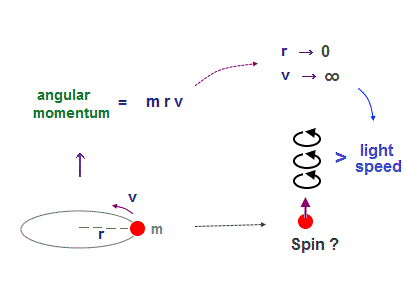Electron spin is faster-than-light rotation, so unreal.

Angular momentum is given by mv × r ( v = velocity, r = radius ).
Electron spin also has angular momentum 1/2ħ, they claim

The problem is an electron is very tiny, point-like.
The point-like particle means its radius r is almost zero.

So to get the angular momentum 1/2ħ, the electron spinning must far exceed light speed ( this p.5 )

So the electron spin lacks reality.
Even Pauli ridiculed the idea of "spinning electron".

But in "s" orbital of Schrodinger's hydrogen, this electron spin is the only generator of magnetic moment.

So they had no choice but to accept this strange spin ( Not as real spinning and speed ).

## Spin has the same Bohr magneton.

### [ Electron spin has the same magnetic moment as Bohr's orbit ! ]

(Fig.7)  ↓ Lucky coincidence ?  Same magnetic moment.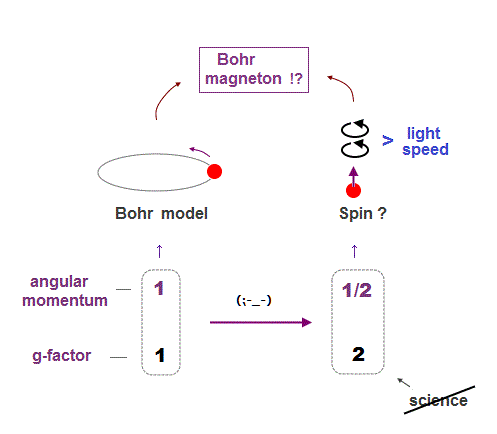It's known that hydrogen atom has magnetism equal to Bohr magneton, which can be explained by Bohr's classical orbit and de Broglie theory.

After quantum mechanics was born, its Schrodinger wavefunction has No orbital angular momentum to explain this magnetism.

So the physicists at the time invented strange spin, and they artificially defined the spin's magnetic moment as the same Bohr magneton !

This is a very far-fetched interpretation.
Spin's angular momentum is 1/2ħ, which is half of Bohr's ħ angular momentum.

So they decided that spin g-factor is twice (= 2 ) the Bohr's orbit (= 1 ).
"g-factor" means the ratio of magnetic moment to angular momentum.

As a result, they claim spin can also has the same Bohr magneton.
We can only measure the magnetism, neither angular momentum nor g-factor.

The problem is there is No physical reason why "spin" cannot stop, and spin has the same Bohr magneton as Bohr's classical orbit.

## Why science stops advancing ?

### [ Technology is great,  but theory is wrong. ]

(Fig.8)   The current technology can manipulate a single atom.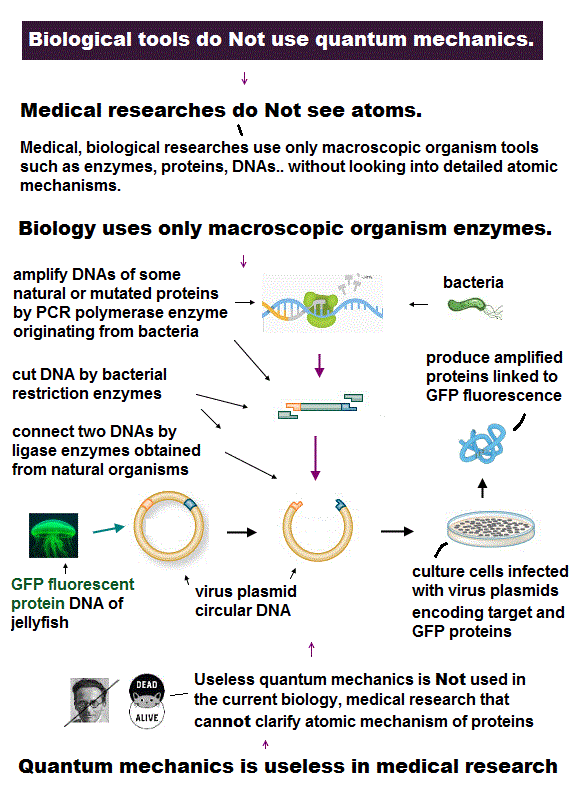We already have technology of manipulating a single atom.

So we should be able to make and manipulate tiny molecular machine to cure fatal disease.  But we cannot.

Basic physics theory, quantum mechanics is too old, with No change for 100 years, which clearly prevents advancing science.

## Anomalous Zeeman effect is not "spin".

### [ Anomalous Zeeman effect is due to inner electrons, not spin. ]

(Fig.9)  ↓ Only sodium shows typical anomalous Zeeman effect.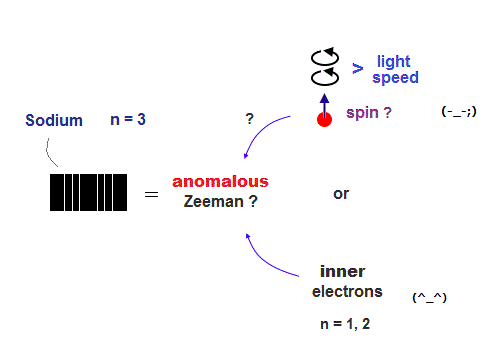Most textbooks say anomalous Zeeman spectrum patterns under magnetic field proved the existence of "spin".  But it's wrong, and disagrees with experiment.

In fact this anomalous Zeeman pattern was seen only in large atoms. Even if you try to find the cases, you can find only sodium case ( this p.3 ).

I bet you can never find similar anomalous Zeeman effect in small hydrogen and lithium atoms, which all show normal Zeeman triplet without spin.

Electron spin lacks reality, its spinning far exceeds light speed c.
Sodium (= Na ) has many inner electrons ( n=1, 2 ), different from one-electron hydrogen.

So it's more natural to think complicated anomalous Zeeman pattern is caused by inner electrons instead of unreal spin.

Furthermore, there is No direct quantitive proof of orbital Lande g factor, which, they claim, is the proof of spin 1/2.

In fact, Zeeman effect under magnetic field is very complicated and depends on freely-adjustable experimental parameters, so it cannot prove the existence of electron spin.

Energy splitting under magnetic field is expressed as complicated " curved lines" called "Breit-Rabi formula".

You cannot isolate only electron spin.  You must mix electron spin and uncertain nuclear spin, introduce mixed artificial parameters ( this p.3  this p.4 )

For example, No experimental data of electron Lande g-factors of alkali atoms ( ex. potassium, this p.9 = gJ values are Not available ).

## Hydrogen is normal Zeeman effect without spin !

### [ H, Li atoms show normal Zeeman triplet (= Paschen-Back ? ) ]

(Fig.10)  One electron H shows "normal" ← Not spin.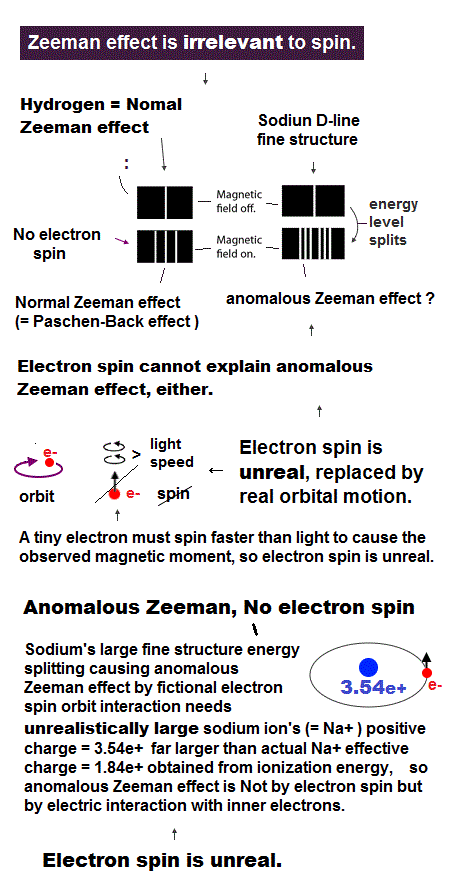Despite textbook's exaggeration of "anomalous Zeeman = spin", one-electron hydrogen shows normal Zeeman effect, which doesn't need spin.

Lithium also shows normal Zeeman triplet pattern.
It is called Paschen-Back effect, which substantially means normal Zeeman.

They claim a hydrogen's small splitting called fine structure is caused by spin-orbit magnetic interaction.  But if it's true, spin-orbit interaction is too small to explain other Alkali fine structure.

So the quantum mechanical idea that fine structure = spin orbit is wrong.

In this book p.659, they say, "for weak magnetic field, each component of hydrogen Hα doublet was separated in normal Zeeman triplet."

This doublet fine structure does not need unreal "spin".
In conclusion, anomalous Zeeman effect in large atoms is not a proof of spin.

## Pauli exclusion principle disproves spin.

### [ Spin magnetic energy is too small to cause Pauli exclusion force. ]

(Fig.11)  Spin-spin magnetic energy (= 0.0001 eV ) is too small !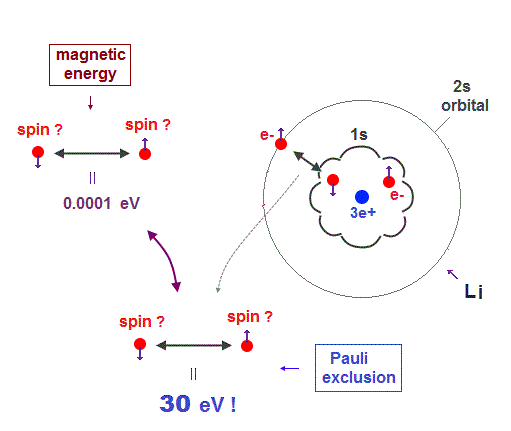Pauli exclusion principle claims that each electron can occupy a different spin-orbital state.  Two 1s electrons of helium must have different spins, up and down.

So the 3rd electron lithium cannot enter the same 1s orbital, because spin states has only two versions up and down.

As a result, this 3rd electron of Li must enter far outer 2s orbital, resisting Coulomb attraction from nucleus.

How strong is this Pauli exclusion force overcoming Coulomb attraction ?
If all three electrons can enter inner 1s orbital, its total energy is 30 eV lower (= stable ) than the actual lithium.

It means "Pauli exclusion force" is as strong as about 30 eV !
But spin-spin magnetic energy is far smaller, only 0.0001 eV ( this p.6 ).

So strong Pauli exclusion principle has nothing to do with spin ( this p.7 ).
Triplet, ferromagnet, molecular bonds are not spin, either.

## Schrödinger equation cannot handle helium.

### [ Quantum mechanics is useless in multi-electron atoms. ]

(Fig.12)  No solution → just "choose" trial functions ! = useless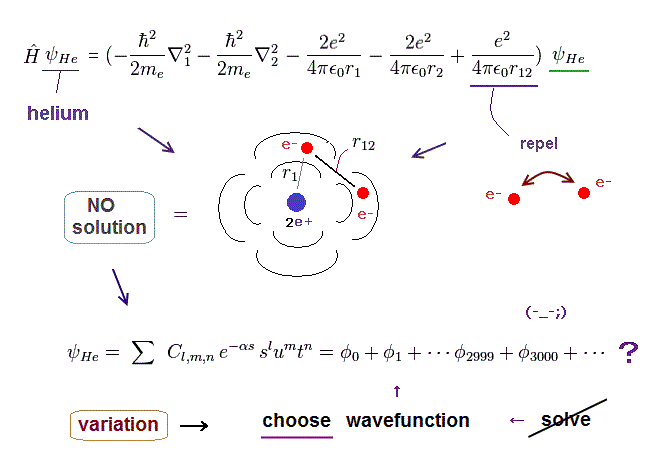Schrodinger equation is false.  Two-electron helium contains interelectronic Coulomb energy.  So Schrödinger equation has No solution of helium.

No multi-electron atoms have exact solution in Schrödinger equation.  Then how does quantum mechanics deal with multi-electron atoms ?

Surprisingly, they just choose artifical trial function as "imaginary" solution.

"Choosing" fake solution and artificial parameters out of infinite choices means Schrödinger equation has no ability to predict multi-electron atoms.

And it's impossible to try infinite kinds of trial wavefunctions and find the one giving the lowest energy in them.

We prove that whatever trial function you choose, you can never get true Schrodinger solution.  So quantum mechanics proves to be wrong !

## Schrödinger calculation of Helium is nonsense.

### [ Cannot solve → choose virtual function = not true energy ! ]

(Fig.13)  "Choose" trial functions → integral over all space. → endlessHere we explain why these "chosen" wavefunction cannot give true ground state energy of helium.

After choosing some trial wavefunction of unsolvable atoms, they integrate them over all space, and get approximate (= fake ) total energy E'.

The point is this approximate energy E' is just an average energy in a collection of different energies depending on different electrons' position.

Originally, the sum of kinetic and potential energy in any electrons' positions must be equal to the single common ground state energy E.

But "unsolvable" multi-electron wavefunctions don't satisfy this basic condition.

So, this "average" energy E' does Not mean the single common ground state energy in any positions of helium.  It causes useless quantum mechanics.

## Why Schrödinger equation is wrong ?

### [ Helium can be divided into three "fake" hydrogens ? ]

(Fig.14)  Helium true energy → three Coulomb terms must be independent !?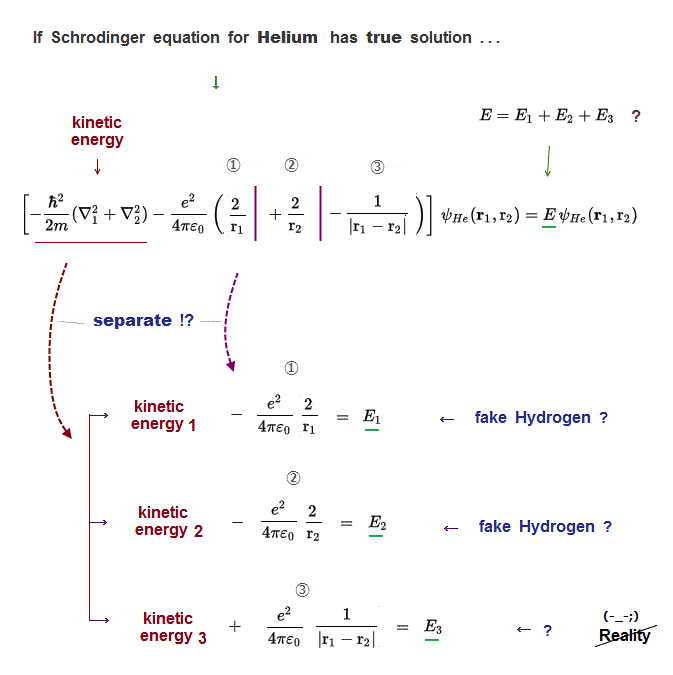If Schrodinger equation for Helium has "true" solution, all three Coulomb energy terms must be completely cancelled out by kinetic energy.

Schrödinger wavefunction always spreads all space.  Its helium approximately consists of two hydrogen wavefunctions, so each electron of helium can move independently everywhere over spreading wavefunction !

It means these three different Coulomb terms must be independently canceled by its corresponding kinetic energy term  → three "fake" hydrogens !?

So in true Helium solution (= if it exists ), Helium can be divided into three "fake" hydrogen, one of which is unreal hydrogen (= ③ is electron-electron hydrogen ? )

It means choosing some approximate function (= basis set ) cannot give true "common" energy, but just "fake" energy.

Instead of Schrodinger's spreading wavefunction, two clear electrons have to classically avoid each other to obey a single total energy in any electrons' positions.

## Density functional theory ( DFT ) is useless.

### [ DFT can freely choose functional which fits experimental result. ]

(Fig.15)  Electron interaction term is freely chosen. ← useless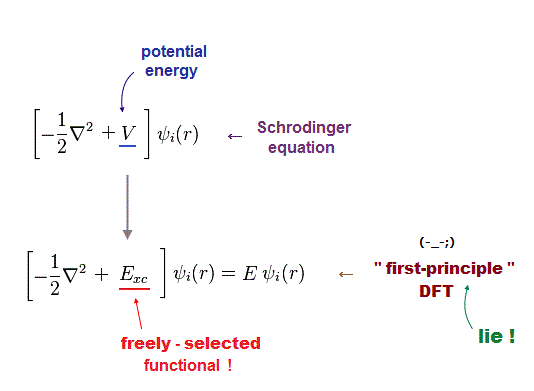In larger atoms in condended matter physics, density functional theory (= DFT ) is the only computing method.

Unfortunately this DFT has No ability to predict any values, so useless.
Like this, DFT just chooses some convenient functional out of infinie choices.

In DFT, "exchange correlation functional" means interelectronic repulsions.  This functional is unknown, can be freely determined ( this p.2 ).

So DFT can be considered a semi-empirical method, different from media-hype ( this p.23 ).

DFT is useless with No ability to predict atomic behavior.

## Electron correlation in DFT is meaningless.

### [ Electron correlation is artificially determined in DFT. ]

(Fig.16)  Exchange, correlation functionals can be "freely" chosen.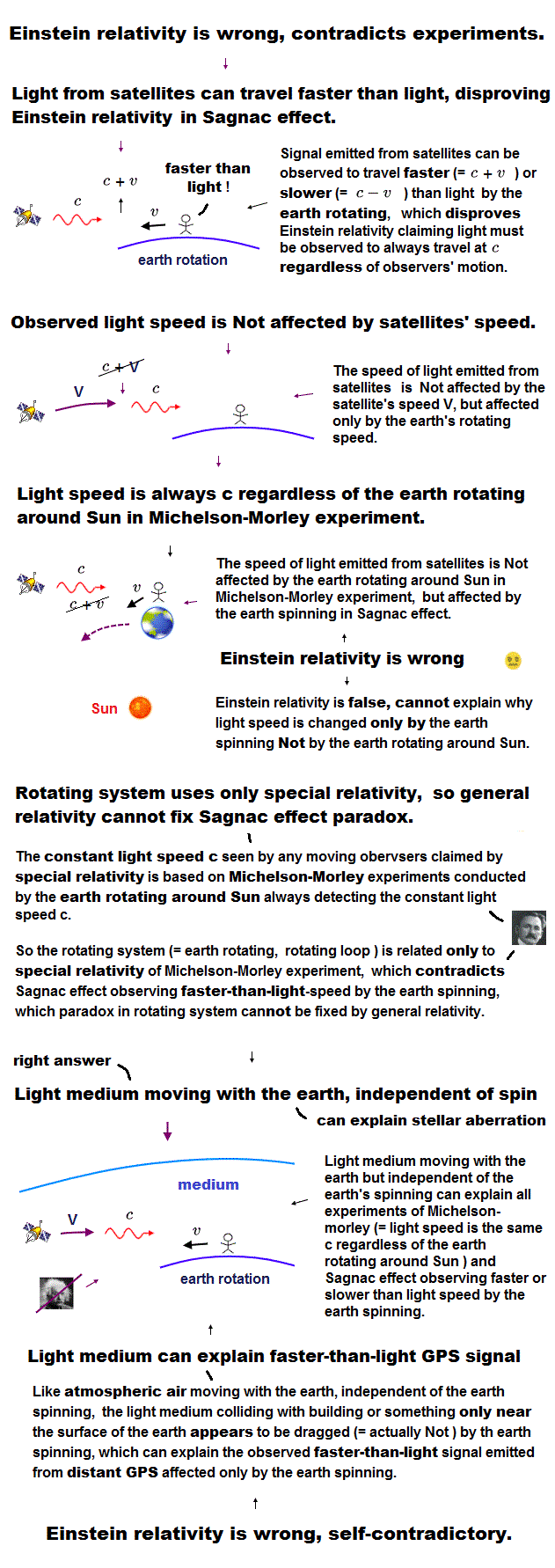The calculated results depend on correlation functionals we choose in DFT.
There is no restriction in choosing these functionals.

No functional is accurate for all properties of interest ( this p.17 ).
No matter what functional is invented, someone will always find a case where it fails.

As you see, quantum mechanics has No ability to predict any energy values.  Molecular mechanics is useless, too.

## Fine structure originates from Bohr-Sommerfeld model.

### [ Bohr-Sommerfeld model agreed with fine structure by Dirac. ]

(Fig.17)  ↓ This was really a lucky coincidence ?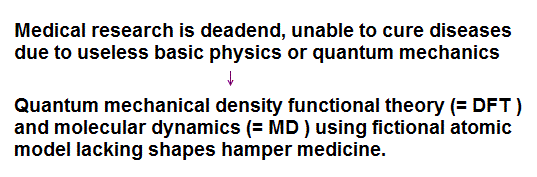The important point is that the fine structure (= small energy splitting ) of hydrogen was first obtained by Sommerfeld using Bohr's orbit.

Later, Dirac equation using spin-orbit interaction got exactly the same solutions as Bohr-Sommerfeld model !  Lucky coincidence ? ( see this last. )

It's surprising that Bohr-Sommerfeld model with No spin gives the same fine structure solution as quantum theory.

Clearly, one of them (= latter Dirac hydrogen ) tried to aim at the same solution as the former Bohr-Sommerfeld, using some trick.

Compare this p.12 and this p.1

This " relativistic effect (= electron's mass becomes heavier, when the electron moves faster ? )" is Not due to Einstein's relativity but due to the original Maxwell theory.

"Atomic bomb proved Einstein mc2" is fake news, too.  Nuclear energy is potential (= Not kinetic ) energy, which means "medium (= rejected by Einstein )" around particles has real mass changed by potential energy which was first shown by Maxwell.

Einstein copied Maxwell mc2 in the wrong way.  Einstein mass is illusion, "A mass can change freely by observer's motion ?"  Physicists still cannot show detailed mechanism of Einstein mass increase or time dilation.

Einstein relativity contradicts the electron's de Broglie wavelength, so Einstein is false.

As an electron moves faster, it generates de Broglie wave of higher energy around it.  This energy of electron's de Broglie wave causes resistance from the medium and "additional mass" to the electron, which agrees with Maxwell's energy mc2, and looks like a heavier electron.

## Lucky coincidences in spin model.

### [ Spin-orbit model contains many lucky energy coincidences. ]

(Fig.18)  ↓ This was really a lucky coincidence ?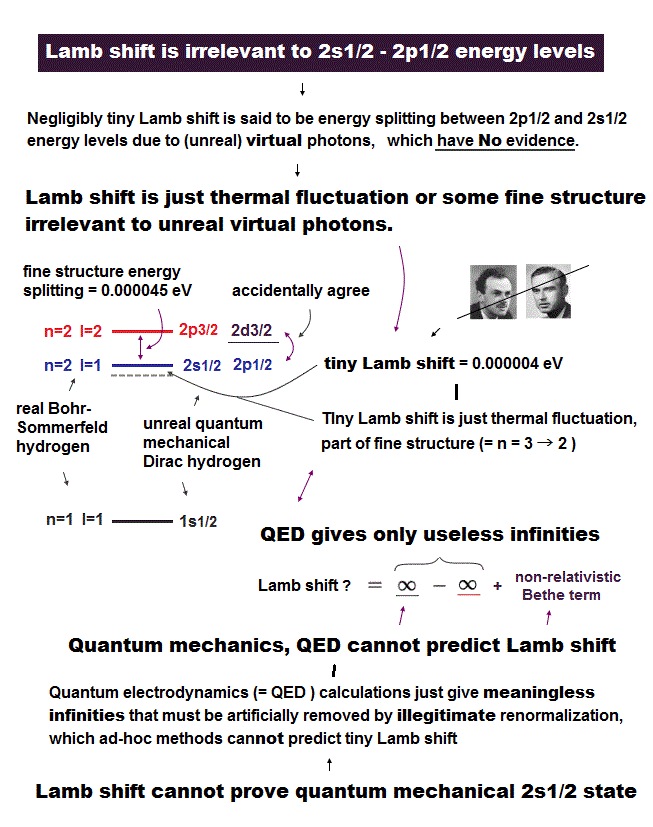Quantum mechanical spin-orbit model should naturally contain much more split energy levels due to its spin, than Bohr-Sommerfeld model.

But hydrogen energy levels are far less than spin-orbit model expected.
The same fine structure solution is gotten from Sommerfeld and Dirac theories.

Because Dirac hydrogen model contains many lucky coincidences in energy levels.

For example, 2s1/2 and 2p1/2 orbitals have the same total energy in Dirac hydrogen, though their figures are completely different.

In the same way, 3s1/2 = 3p1/2,  3p3/2 = 3d3/2,  4s1/2 = 4p1/2 ...
As you see, the present spin-orbit model relies on very unnatural coincidences.

Bohr-Sommerfeld fine structure is more natural and correct than artificial Dirac equation !

## Relativistic Dirac equation with spin ?

### [ Einstein mass relation → Dirac equation with spin σ ? ]

(Fig.19)  ↓ Linear Dirac equation contains spin ?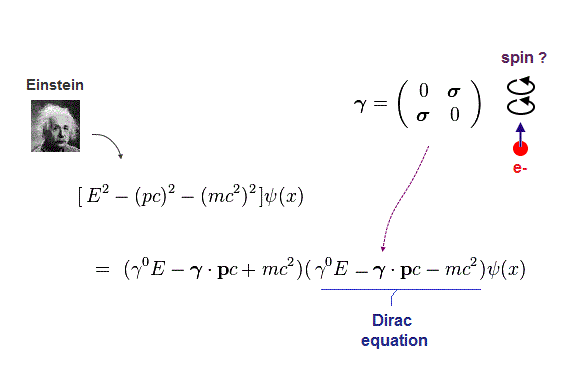In fact, Quantum field theory lacks reality, where Dirac equation was gotten by dividing Einstein quadratic relation into linear functions.

In compensation for linear function, Dirac equation must contain 4 × 4 gamma (= γ ) matrices, which consist of spin Pauli matrices (= σ ).

This is the reason they claim Dirac equation succeeded in combining "spin" and relativity.  But "σ = spin ?" is just artificial definition with No grounds !

To begin with, these Pauli σ matrices are just the result of changing quadratic → linear functions. They have nothing to do with unreal spin

Furthermore, Einstein mc2 causes serious paradox.

## Spin-orbit coupling is Einstein relativity ?

### [ Fine structure = relativistic spin-orbit interaction is wrong. ]

(Fig.20)  ↓ H atom fine structure is relativistic effect ?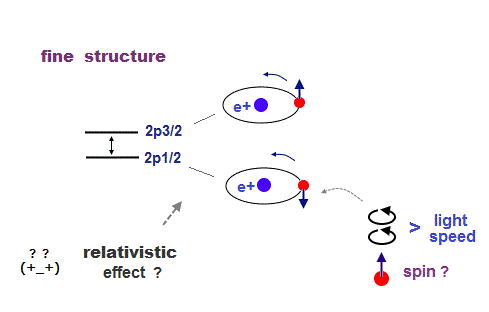Hydrogen atom has small energy splitting (= fine structure ).
They say this splitting shows the difference between electron's spin up and down.

This spin-orbit interaction is said to be Einstein relativistic effect.
In H atom, an electron is moving around a proton (= nucleus ).

From the electron's point of reference, the proton appears to be moving.
Einstein relativity is based on purely relative ( not absolute ) motion.

So even if the proton is actually stationary, the electron feels the pseudo-magnetic field created by moving proton, which causes small energy splitting depending on spin direction ?

The point is this relativistic electromagnetic fields cause fatal paradox of Lorentz force !  So spin-orbit coupling model is completely false with flaw.

## Na fine structure is too big for spin-orbit.

### [ Large Na fine structure splitting is impossible by Na+ charge. ]

(Fig.21) Na+ ion must have 3.5 positive charge, if spin-orbit is true.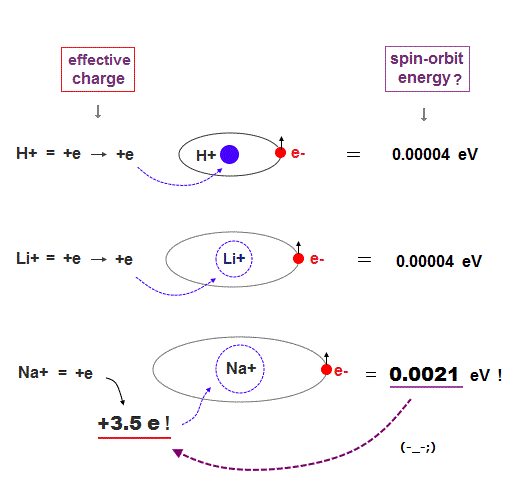They say Na large fine structure in D lines is also due to spin-orbit interaction.  The problem is this Na fine structure splitting is too big.

Compare fine structure splitting in H (= 0.000045 eV ) and Na (= 0.0021 eV ) atoms.

It is known that this fine structure splitting is proportional to Z4/n3, where n is principal quantum number.  See this last and this p.2.

Z is effective positive charge (= H+, Na+ ion ), which movement causes magnetic field at the electron's spin ?

To get the large Na fine structure, this central charge (= Na nucleus + all inner electrons ) must be unrealistically big ( Z is +3.5 ).

In other alkali atoms, the situation becomes much worse.
So relativistic "spin-orbit" interaction is too weak to cause alkali fine structure !

It means fine structure energy splitting is caused Not by spin-orbit interaction, but by other Coulomb effect or something.

## Electron de Broglie wave was confirmed.

### [ de Broglie relation was confimed in various experiments. ]

(Fig.22)  Double-slit experiments proved electron's de Broglie wavelength.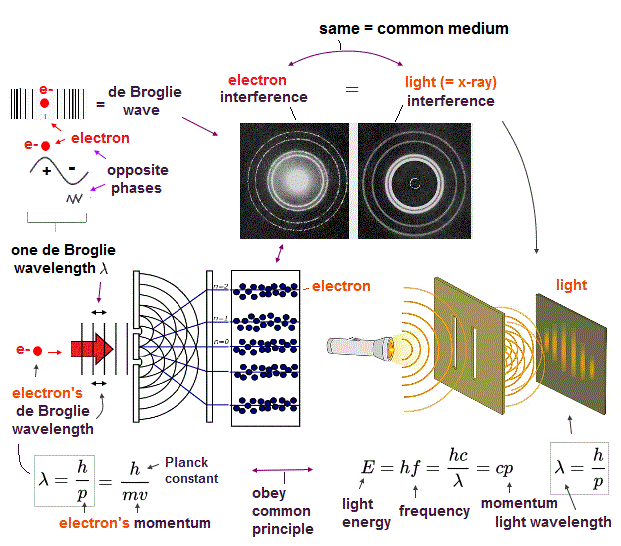In de Broglie relation, electron's wavelength λ is given by λ = h/mv, where m and v are electron's mass and velocity.

This important matter-wave relation was confirmed in various experiments such as doube-slit, Davisson-Germer and this.

So there is No room for doubt that this de Broglie wave is true.

In these experiments, an electron is driven out from destructive interference regions by the "force" of de Broglie wave ( this p.25 )

This strong "expelling force" by de Broglie wave interference can explain strong Pauli exclusion force !

## Schrödinger "distorts" de Broglie relation.

### [ Quantum theory uses de Broglie relation, but "distorts" it ! ]

(Fig.23)  Quantum mechanical wavefunction is unreal.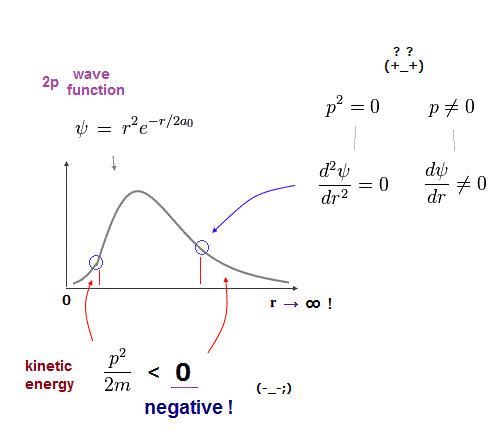So Schrodinger equation adopted this de Broglie relation as "derivative" form.
Momentum operator (= derivative of wavefunction ) links p and λ.

Of course, when momentum p is zero, its square p2 must be zero, too.
But only when a wavefunction has basic " cos" or "sin" form, it holds true.

The point is quantum mechanical wave functions distort original de Broglie relation.  Figure above is hydrogen 2p radial wavefunction ( this, this last ).

"2p" wavefunction has unreal negative kinetic energy on both sides.

On these boundaries, the second derivative is zero ( p2 = 0 ), but first derivative is not zero ( p is not zero ) !  This is ridiculous.

It's quite natural that when p is zero, its square p2 is zero, too !
So quantum mechanics distorts original de Broglie relation, and uses wrong math !

## Schrödinger and de Broglie wave

### [ Schrödinger hydrogen obeys an interger times de Broglie wave ! ]

(Fig.24) Schrodinger's orbital is n × de Broglie wavelength.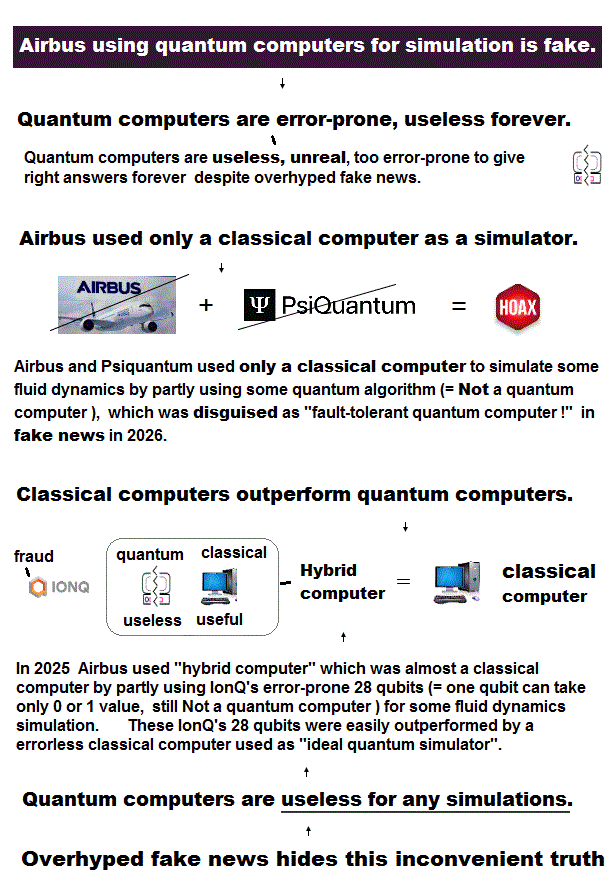Historical magic shows Bohr model agreed with experimental results and Schrodinger's hydrogen using de Broglie theory.

Bohr model's orbit must be an integer times de Broglie wavelength.
Then, Schrodinger's hydrogen also obeys "an integer times de Broglie wavelength" ?

In fact, Schrodinger orbitals also meet an integer times de Broglie wavelength like classical quantum theory ( this last ) !

"Boundary" condition at both ends ( r= 0,∞ ) in Schrodinger hydrogen corresponds to de Broglie condition ( see this p.11, 12 ).

When we use u = rR as radial wavefunction, de Broglie relation is clearer ( this p.3 ).

As you see, Schrodinger hydrogen clearly obeys "n × de Broglie wavelength" !
But its angular momentum = zero is contradictory, so useless as precondition.

## Old Bohr model failed in three-body helium.

### [ It was impossible to obtain correct three-body helium model in 1920's without computers. ]

(Fig.25)   Simple circular old Bohr's helium gived wrong energy (= -83.33 eV ).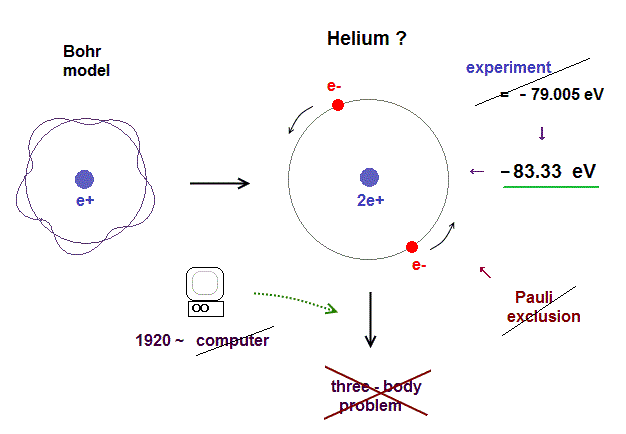The most decisive reason for dismissing Bohr model is failure in explaining helium atom.

As shown in this section, simple circular helium model above right gives wrong ground state energy (= -83.33 eV ).

The helium experimental value is -79.005147 eV (= 1st + 2nd ionization energies, Nist, CRC ).

Of course, there were NO convenient computers in 1920s to simulate three-body motions (= two electrons + one nucleus ) like helium.

On the other hand, quantum mechanical variational methods can get approximate helium energy, though it does Not mean truth.

Even wrong approximate solution was far better than the dire situation where physicists had nothing to study without computers in realistic orbits in 1920s.

So this lack of computers dealing with three-body atoms is the main reason we had gone the wrong way.

## A single electron interference = parallel worlds ?

### [ Real electron with de Broglie wave is needed. ]

(Fig.26)  A single electron splits into parallel worlds !?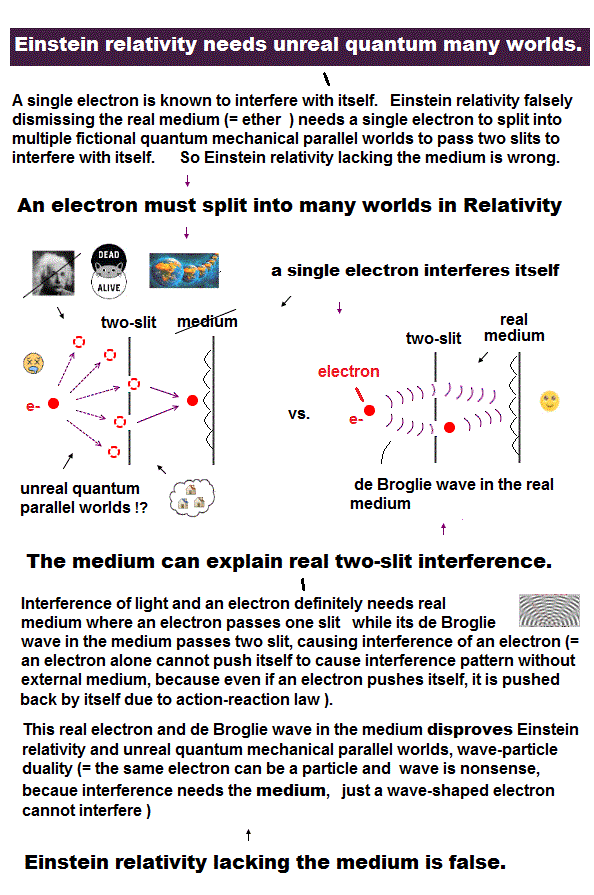Even a single electron can interfere with itself in two-slit experiment.

Quantum mechanics says an electron can pass both two slits simultaneously in two parallel worlds !  This is unrealistic.

We can naturally explain this double-slit using real electron with de Broglie wave interference.  We don't need parallel worlds !

Fantasy parallel worlds originates from Einstein denying medium.
Einstein relativity cannot explain light refraction and paradox.

So quantum mechanics and Einstein relativity are the main reason why they adopt unreal parallel worlds in double-slit experiment.

## Photon is just electromagnetic wave.

### [ Electromagnetic wave of 1 km wavelength = a photon ? ]

(Fig.27)  ↓ A single photon is bigger than 1000 meter !?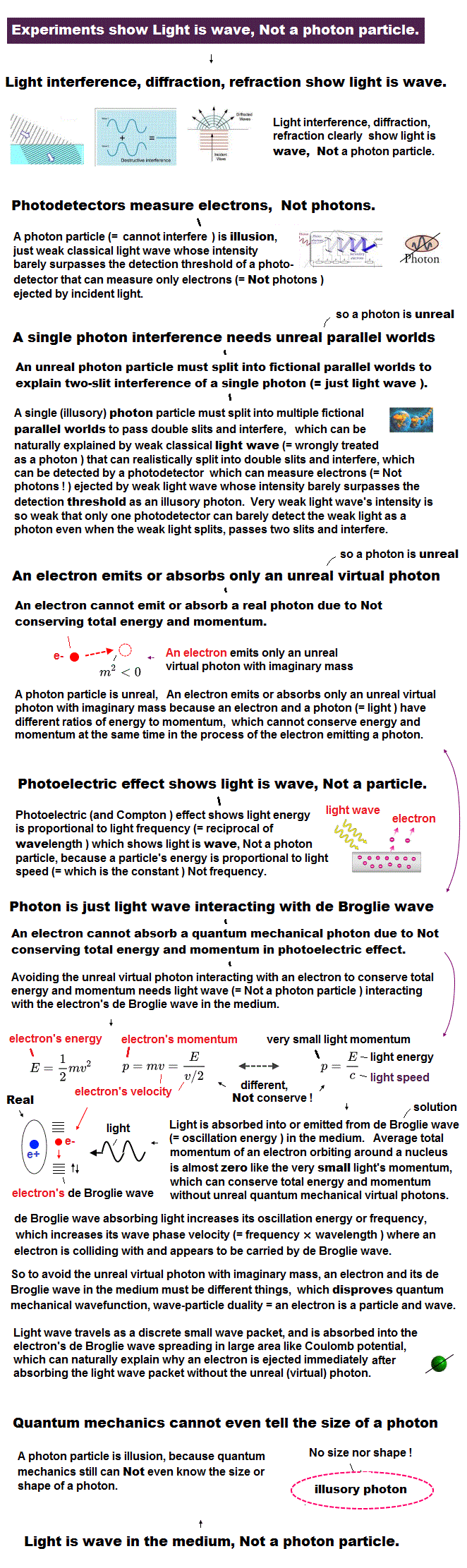You may often see "photon", a quantum particle of electromagnetic wave ? in various academic sites and news.

OK. Then how big is a single photon ?
In fact, the present physics cannot answer even this basic question !

For example, radio wave is one of electromagnetic waves, which have very long 1000 meter wavelength.  A single photon is so big ?

We have never confimed so big photon.  So a photon is just a fictional particle.
Even Nobel laureate, Lamb (= photon experimentalist ) did Not believe a photon.

## Photoelectric effect is NOT a proof of photon !

### [ Threshold light frequency (= f ) means light is "wave". ]

(Fig.28)  Electron is ejected above some light frequency.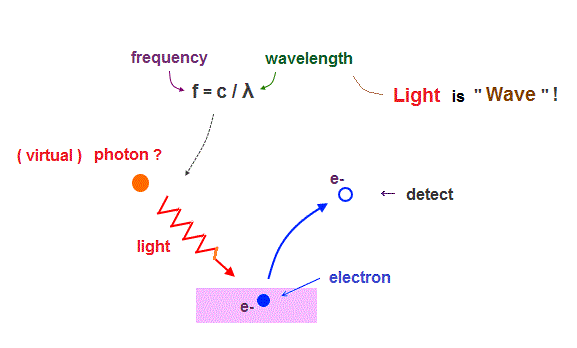Quantum mechanics claims Einstein's photoelectric effect proved a photon.
But I bet you won't be able to find any clear photon images in them.

The point is in photoelectric effect, all you can detect is electrons ejected by light (= photon ? ).  No photons can be seen directly.

When you shine light above the threshold frequency (= f ) on to a metal, electrons are emitted from the metal, which is detected.  That's all.

As you see, there is No proof of a photon particle here.
Light frequency is equal to c / wavelength, which means "wave" !

So this famous photoelectric effect just showed incident light is "wave having frequency", Not a photon particle.

A photon interacting with a electron must be "virtual", not real.
Photon particle cannot explain light refraction.

## Photodetector detects "electrons" Not photon.

### [ A single photon detector measures ejected electrons, Not a photon ! ]

(Fig.29)  Increased ejected photoelectrons = a photon ?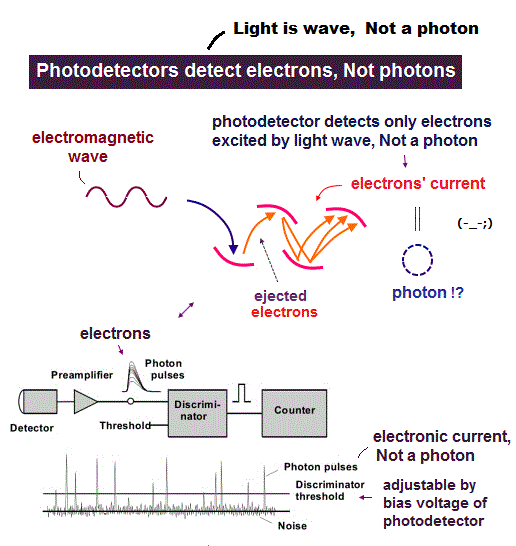Then what is a "photon" in university and the media based on ?
They claim a single photon detector can detect each photon particle ?

Again, this explanation is misleading.  Because a single photodetector detects Not a photon, but electrons' current excited by incident light.

Only when frequency and intensity of an incident light exceed some threshold (= which can be adjusted ), they call its electric signal "photon".

So there is No experimental proof of a photon.

Fictional photon is needed only for publishing journals and the current useless quantum theory.

## Accelerating electron radiates energy ?

### [ A 'single' moving electron doesn't lose energy. ]

(Fig.30)  An electron emits and loses energy ?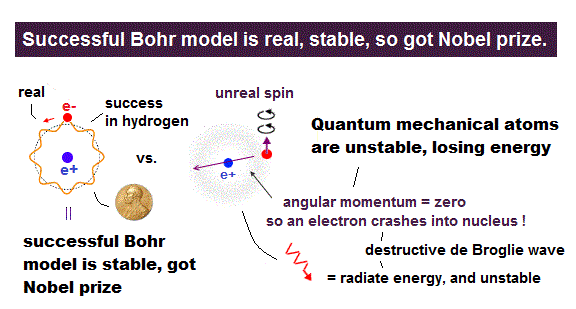You may often see the boring cliche "accelerating electron radiates energy in classical orbit", so Bohr's hydrogen is unstable.

This logic is wrong.  So Bohr's orbit is stable, Not radiating energy.

The right answer is "a single accelerated charge ( like in Bohr's hydrogen ) does Not radiate energy."

Only when many electrons are accelerated and colliding with each other disorderly as seen in alternating currents, they radiate and lose energy, thereofore we need to constantly supply electric energy for them to radiate electromagnetic (radio) waves.

So the "accelerating" electron losing energy does Not apply to the Bohr model where an electron is moving in an orderly and stable way conserving total energy between a nucleus and an electron, avoiding destructive interference of de Broglie wave.

## Bohr's electron does NOT radiate energy.

### [ When an electron is a spherical conductor consisting of "many smaller charges", it loses energy. ]

(Fig.31) Bohr model electron is Not falling into nucleus.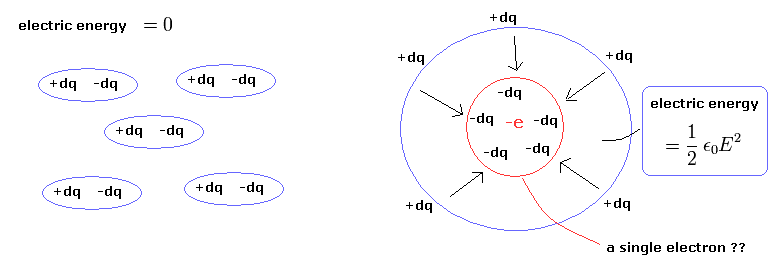In fact, if an accelerating electron loses energy, the single electron must consist of many smaller charges as seen in a spherical conductor.

When Coulomb potential energies among those many smaller charges inside a single electron decrease ( due to repulsions among smaller negative charges ) in a disorderly accelerating movement, it can lose energy, which doesn't apply to Bohr model.

Because if a single electron consists of many smaller charges, the electron easily breaks into many smaller charges due to their Coulomb repulsion from the beginning, which is Not happening as seen in a very stable, unbreakable single electron.

So in a stable single electron as in Bohr model, we don't need to consider repulsions among illusory smaller charges inside one single electron, therefore Bohr's accelerating electron doesn't lose energy.

They uses Poynting vector (= E × H ) as the energy flow ( this, this ), which is equal to the change of the electric and magnetic energy densities stored in the vacuum.

This stored energy (= 1/2εE2 ) means the potential energies needed to gather infinitesimal charges to the spherical conductor

So, if there is Only a single electron, these electromagnetic energies cannot be generated from the beginning !

Also the magnetic energy they use is "stored energy in solenoid", which needs more than one charges !

So using only a single accelerated electron as an example of radiating energy is nonsense and wrong ( this p.4 ).

## A electron cannot emit a photon !

### [ Photon cannot conserve total energy and momentum. ]

(Fig.32)  ↓ A electron radiates a virtual photon with negative mass !?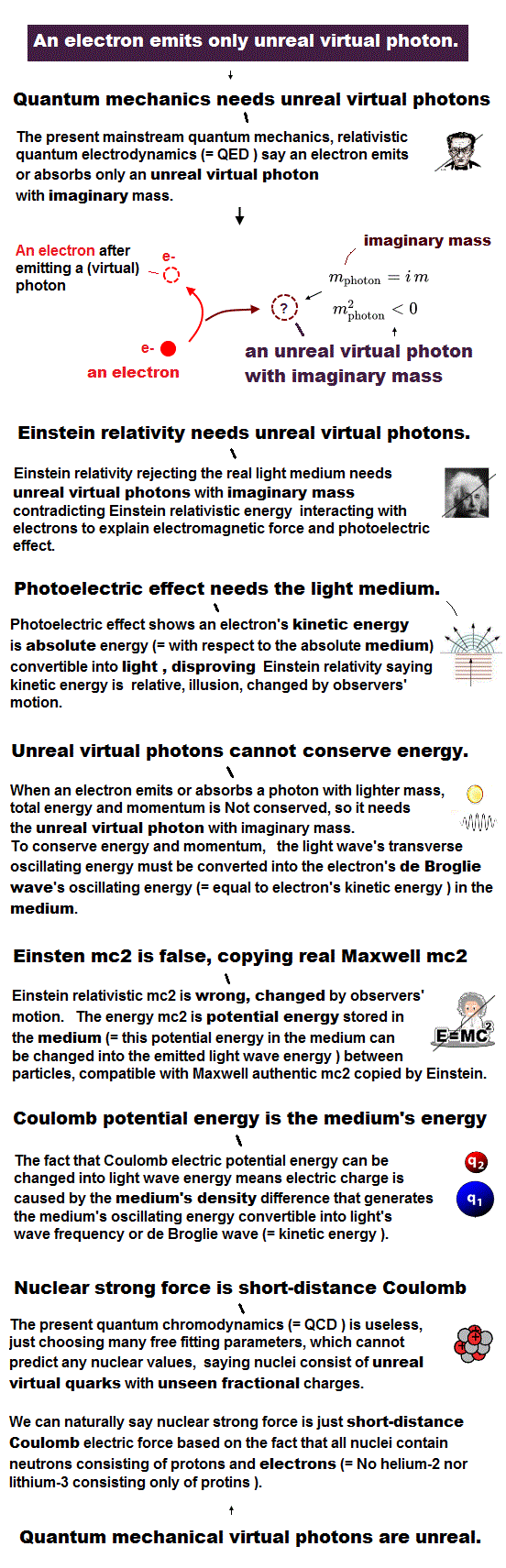In fact, it's impossible that a single electron emits a real photon, because total energy and momentum are Not conserved.

If total energy and momentum are conserved between an electron and photon, this photon must be unreal virtual photon with negative mass squared ! ( this p.3 )

The light (= photon ? ) has an energy proportional to its frequency as E = hf, and its momentum p = E/c.

An electron has its momentum p = mv and its kinetic energy E = 1/2mv2.
Suppose this electron emits a photon and loses its kinetic energy.

Of course, total energy and momentum must be conserved.
But it's impossible to satisfy both energy and momentum conservation !

In general, when a particle emits ( or absorbs ) another particle of different mass, one of energy or momentum is Not conserved.

Because in a photon with No mass, its momentum is much smaller compared to its great energy.

So only Compton scattering without emitting or absorbing is allowed.
Or the whole orbit of an electron and nucleus must emit electromagnetic wave.

## The present physics relies on unreal particles.

### [ Particle physics cannot avoid "unreal" virtual particles. ]

(Fig.33)  ↓Coulomb, Higgs depend on fictional virtual particles.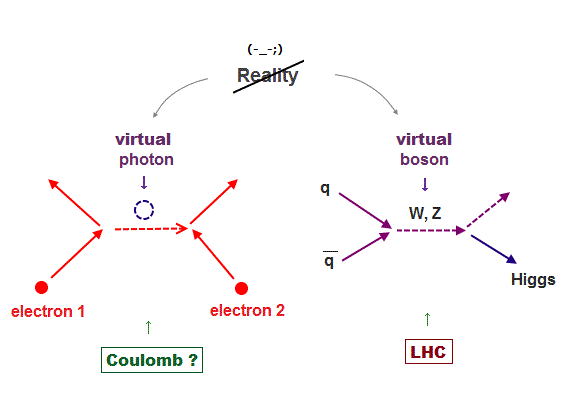In fact, the present physics completely depends on unreal virtual particles as four fundamental forces.

All particle physics reactions need virtual particles, too.
Even Coulomb force depends on unreal virtual photon.

What is "virtual particle" ?  Move faster than light ?
In beta and Higgs decay, Weak boson is virtual, disobeys Einstein mass formula.

So the current physics is unrealistic and wrong.

## Ferromagnet has nothing to do with spin.

### [ Spin magnetic moment is too weak to explain ferromagnetism. ]

(Fig.34)  Spin magnet is too weak to explain ferromagnet.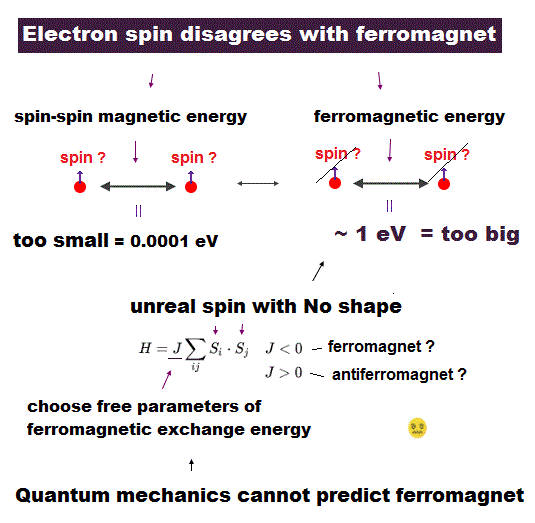Electron spin lacks reality !
Its spinning far exceeds light speed.

Spin-spin magnetic interaction is too weak to explain actual ferromagnet.  See this p.6 this p.7.

Ferromagnetism needs strong interaction between atoms (= orbital motion + Coulomb ) other than unreal weak spin.

Then, what the heck does this spin model mean ?
It uses old "Heisenberg" spin model ( this p.3 ).

But this Heisenberg spin model is too old, which was introduced in 1920s, and it's too abstract to describe actual phenomena ( this p.2 ).

Electron spin is unreal, too small to explain ferromagnet, and too abstract to be useful.

## Quantum mechanics is useless.

### [ Useless quantum mechanics spawned "imaginary" targets. ]

(Fig.35)   Quantum equation cannot handle multi-electrons.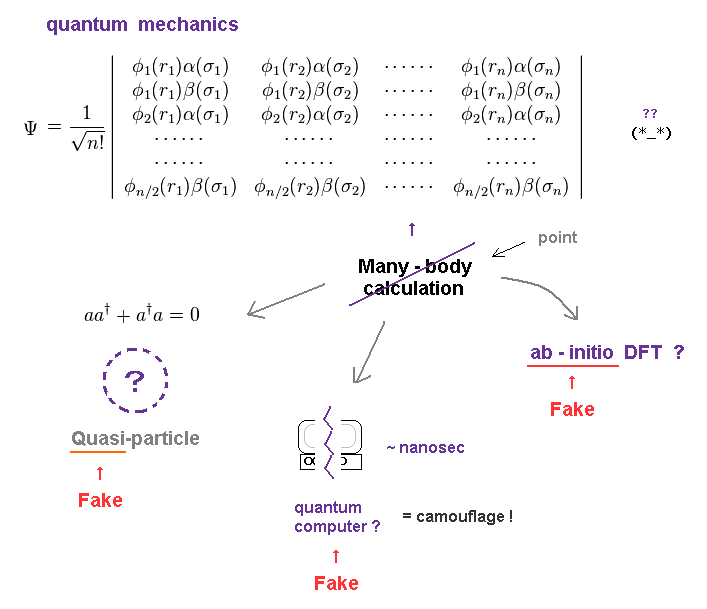Schrödinger equation in quantum mechanics is useless, cannot predict atomic behavior.

So the present physicists give up clarifying true microscopic mechanism.

They focus on fabricating unreal quasiparticles or quantum computer scam to deceive taxpayers and governments.

## Physics is filled with unreal quasiparticles.

### [ Quasiparticle has fake mass and charge. ]

(Fig.36)   Quasi-particles will remain "fake" forever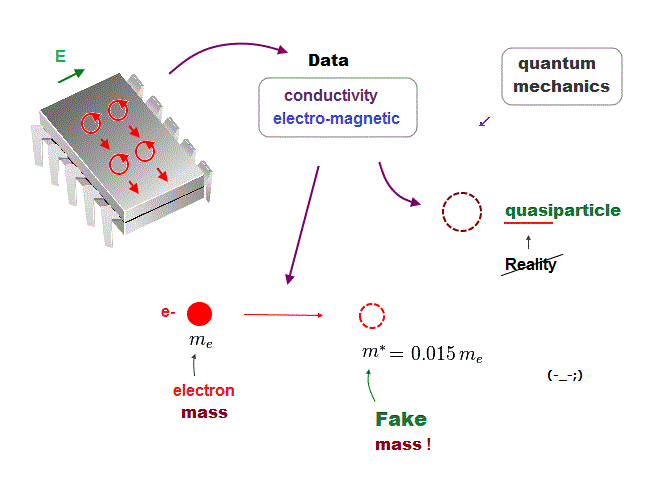Quantum mechanics has No ability to describe various physical phenomena using real objects.

According to Nature, a fundamental electron can split into three components such as "spinon" (= spin ! ), holon (= charge !) and orbiton (= orbital motion ! ).

Of course, these are fictitious (= unreal ) quasi-particle.
These fake quasi-particle cannot exist independently outside the material.

The current condensed matter physics just focuses on creating fictitious quasi-particle, instead of clarifying true underlying mechanisms.  ← nonsense.

Quasiparticle is unreal with fake mass and charge.

## Condensed matter physics.

### [ Quasiparticle is just a "trick" using meaningless symbols. ]

(Fig.37)  Quasiparticle = trick with nonphysical symbols.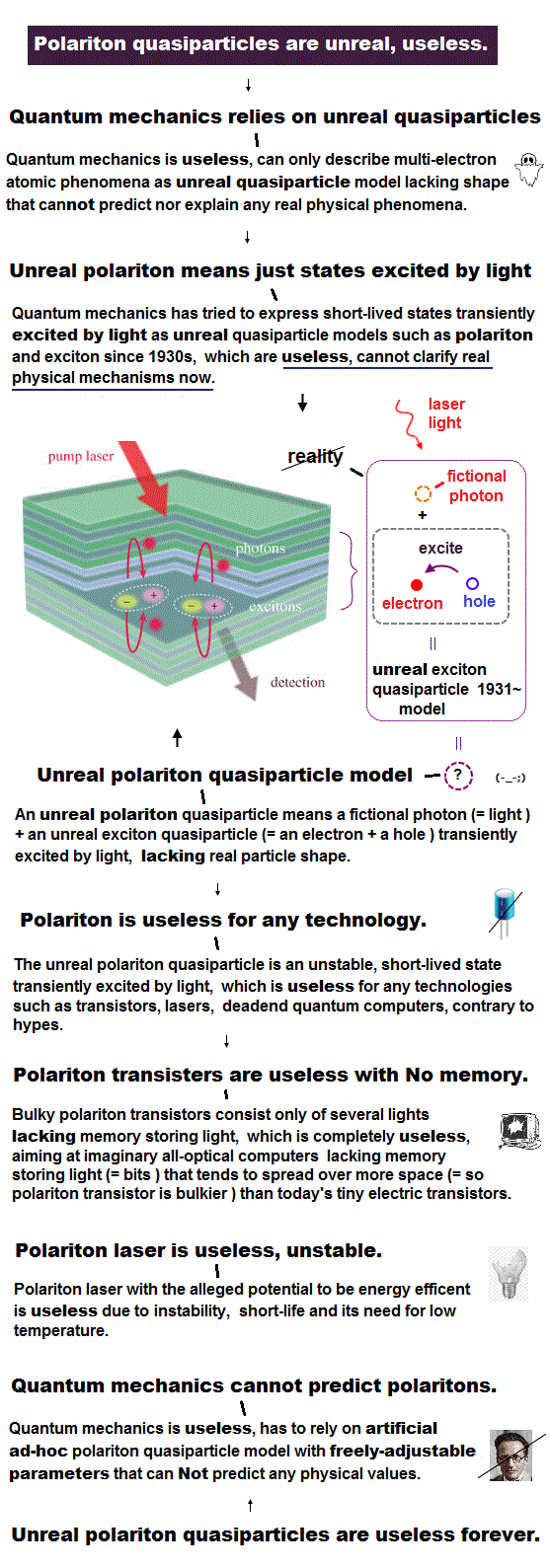A recent journal still deals with unreal quasiparticle, exciton.
So the present science stops in condensed matter physics.

Quasiparticle "exciton" is just a pair of electron (= c ) and its hole (= β ).
NO physical shape in this nonphysical symbol ( this p.3 ).

Polariton is also unreal quasiparticle.  Polariton consists of a pair of exciton and photon ( this eq-(15) , this ).  That's all. It's not a modern physics !

In this way, all the present physics can do is make unreal quasiparticles ( this, this ).  So useless.

## Superconductor by quantum mechanics is unreal.

### [ Superconductor model relies on unreal quasiparticle, so useless. ]

(Fig.38)  ↓Bogoliubov quasiparticle lacks reality.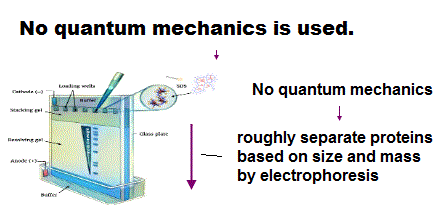The present superconductor model relies on unreal phonon quasiparticle, and fictitious Bogoliubov quasiparticle.

Relying on "not actual" quasiparticle means the present science stops pursuing the truth !   It hampers all applied science.

Furthermore, this Bogliubov quasiparticle contradicts normal particle.
This quasiparticle consists of creation and annihilation of electrons ( this p.4 ).

So "create + annihilate = zero" is what this quasiparticle is !
The problem is the current superconductor model stops at old BCS model, forever.

## Quantum computer = Parallel worlds ?

### [ Quantum computer is uselsss, just "science scam" ! ]

(Fig.39)  Parallel computing using parallel worlds ?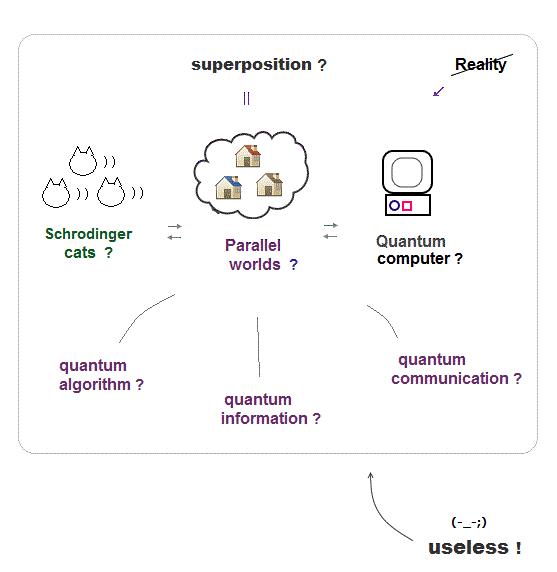Quantum computer is fantasy.  They say it can compute different things simultaneously using unreal parallel worlds.

But there is No evidence of fantasy parallel worlds nor faster quantum computer.

Quantum computer is impractical forever, so science scam.

## Quantum computer is "scam".

### [ Corporations and governments are wasting money in fantasy ! ]

(Fig.40)  Quantum computer (= parallel worlds ) vs. d-waveQuantum computer is said to be faster exploiting fantasy parallel-world computing.  But there is No evidence of faster quantum computer.

Quantum computer is one of the biggest science scams where all corrupt academia, corporations, media collude to defraud taxpayers of money.

IBM and Intel's 50-bit quantum computer is still theoretical, Not viable.   D-Wave 2000-bit computer is Not quantum computer, Not faster.

They say each quantum computer bit can be two different energy states simultaneously using two parallel worlds like a dead and alive cat.

This is untrue.  They are just alternating between two states (= Not simultaneous ! ).
Fraud quantum computer is an easy way to defraud taxpayers of money.

## Particle is just an abstract symbol ?

### [ The present physics can only create or annihilate particle. ]

(Fig.41)  ↓ Electron, photon are just meaningless math symbols.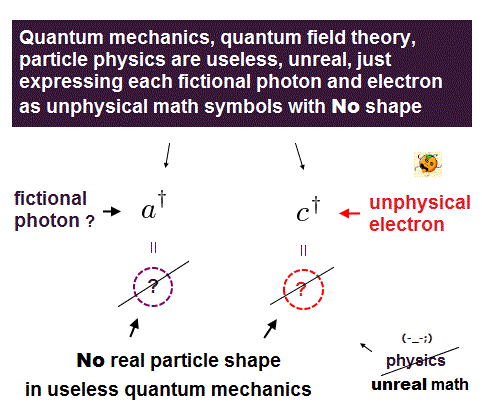What figure does each electron and photon have ?
Unfortunately the present physics has No ability to describe it.

Electron and photon are just abstract math symbols with No shape.
Quntum field theory is based on quantum mechanics and special relativity.

In this theory, all physicists can do is two simple actions; create or annihilate each particle.  That's all.

So this useless physics clearly prevents all applied science from developing, and is harmful to all science students.

## "Anticommute" means Pauli exclusion ?

### [ The present physics cannot give No detailed Pauli mechanism. ]

(Fig.42)  Pauli exclusion principle is just anticummutation ?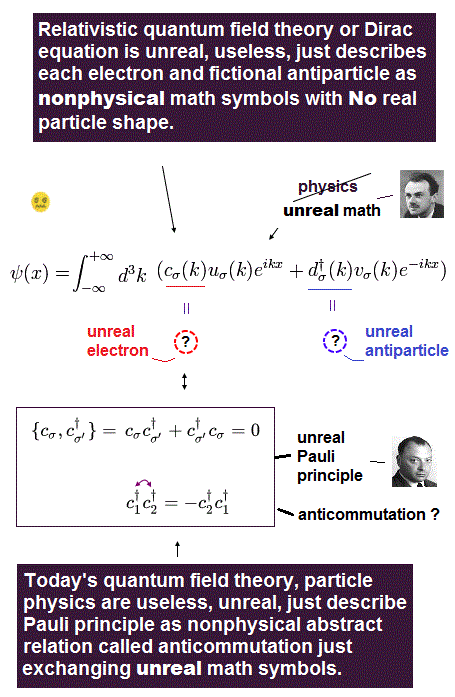Pauli exclusion principle claims that two electron cannot have the same state with regard to orbital and spin.

In fact, electron spin magnetic energy is too weak to explain Pauli strong exclusion force.  So quatum mechanicsl spin is wrong.

Pauli principle. by quantum mechanics is too abstract and nonphysical.
Dirac combined "spin" and special relativity to derive Pauli exclusion.

The problem is in his relativistic theory, each electron is just a nonphysical symbol (= a ) with no shape, and having flaws.

So this theory just says Pauli exclusion is due to abstract anticommutation of fermions' operators.  No detailed mechanism is mentioned.

As shown the above figure, if two electrons are the same ( c1 = c2 ), these electrons are zero ?  ← Pauli exlusion ?  This too simple model explains nothing.

## How an electron emits a photon ?

### [ An electron is annihilated, a photon is created ?  ←NOT physics ! ]

(Fig.43)  Only particle creation and annihilation in photon emission ?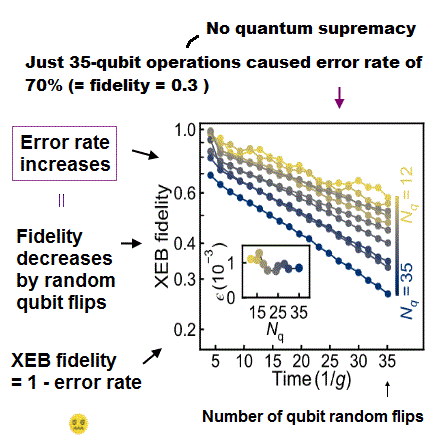All quantum mechanics can do is two simple actions: create or annihilate each particle.  Electron is expressed by Dirac fields (= ψ ), and photon is Maxwell equation (= A ).

When an electron emits a ( virtual ) photon, incident electron is annihilated, a photon is created, and outgoing electron is created.  That's all they can express. So, useless !

This process is one simple interaction term ( this, this ).  Dirac and Maxwell fields in this term include creation and annihilation operators of electron and photon.

Quantum mechanics says No more detailed mechanism about electron emitting photon than this !  ← useless quantum mechanics.

## Particle physics is unreal.

### [ Higgs, quarks .. through Dirac equation are all unreal. ]

(Fig.44)  Einstein mc2 = paradox → Dirac equation → unreal particles !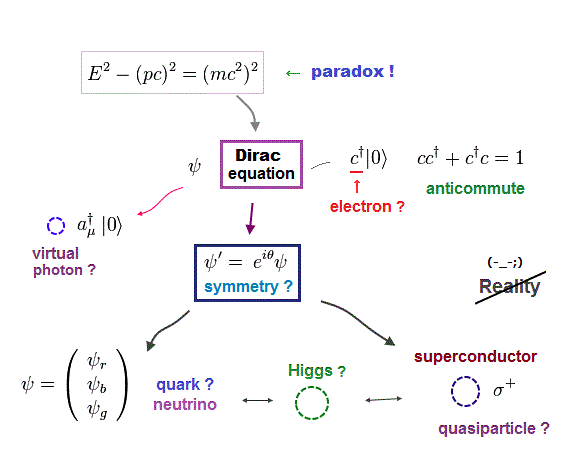They developed Einstein mc2 to quantum field theory, which lacks reality, only describing each particle as abstract nonphysical math symbol.

Quantum field theory uses unreal virtual particles as interacting particles, and all particles inside collider are unstable and unreal.

They suddenly started to claim out nature obeys some "symmerty", but later its symmetry is broken !  So "symmetry" is meaningless.

All these mess originates from the fact Einstein mc2 is illusion due to its serious paradox.

## Pursuing  unreal particles.

### [ Unreal elementary particles cannot be detected directly. ]

(Fig.45)  Fractional charge quark, Higgs field cannot be detected !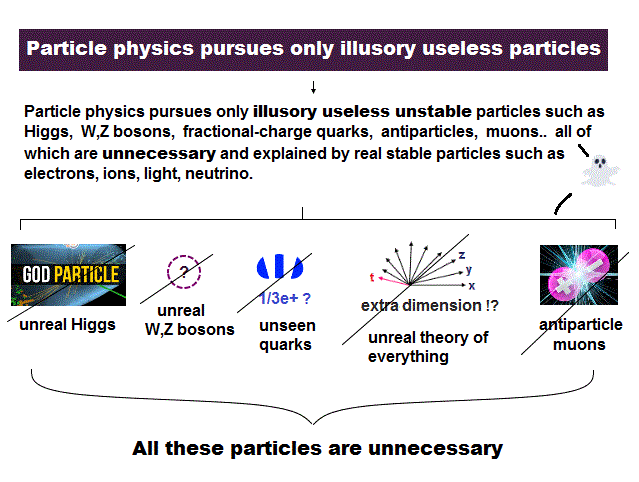The present particle physics seeks only unreal particles.

Fractional-charge quarks can neither be separated nor confirmed.
Weak-force boson is virtual, unreal and violating conservation law.

Higgs field giving mass to everything cannot be detected in our daily lives !  Doubtful Higgs boson is too unstable to observe directly, too

There are the same amount of supersymmetric particles (= SUSY ) around us ?  But SUSY cannot be detected except as suspicious "missing energy".

The present physics seeks only undetectable imaginary particles, which cause fantasy theory.

## Standard model is Not physics !

### [ "Symmetry" in particle physics is nonsense. ]

(Fig.46)  "Symmetry" has NO physical meaning, so Higgs is unreal.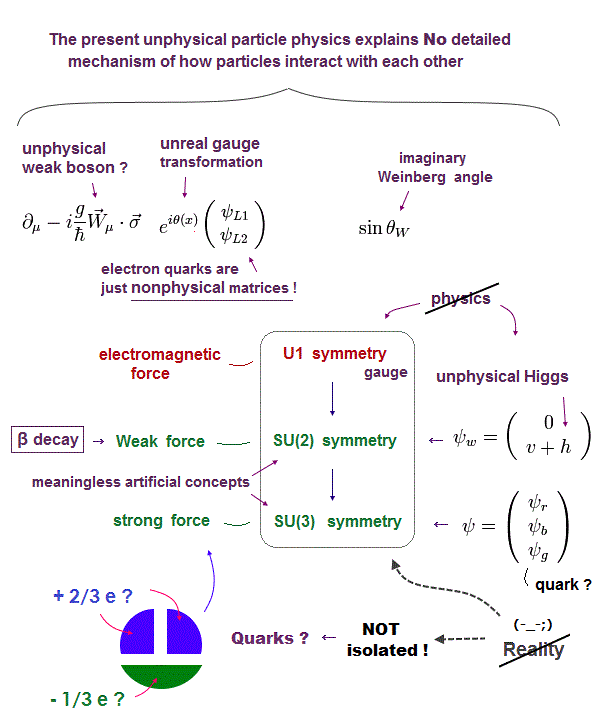Particle colliders such LHC just waste taxpayer's money, they pursue unreal particles which are too short-lived to confirm their reality,  useless for us.

Physicists suddenly introduced artificial baseless rule symmetry and nonphysical gauge but later said "symmetry is broken!"  ← nonsense !

They started to say unreal weak and strong force are SU(2,3) symmetry which is just nonphysical math.

All these doubtful particles such as Higgs, Weak boson, quarks remain useless, just waste money in gigantic colliders.  So they are unreal.

## Light speed c is constant.  → Einstein ?

### [ Michelson-Morley experiment didn't deny light medium. ]

(Fig.47)  Light medium moving "with" the earth is OK ↓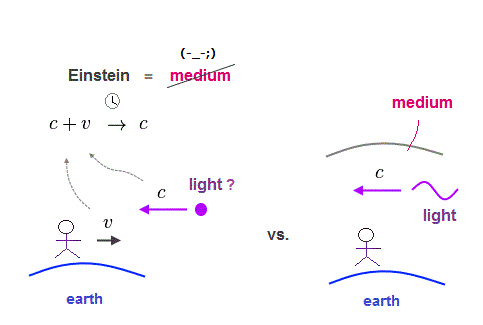Einstein is false.

Michelson-Morley experiment showed that light speed is constant c, which agrees with light medium moving with the earth.

Einstein rejected "medium", so his relativity causes occult phenomena that clock time of moving object is slower.

But from moving object, stationary clock is moving, so we don't know which clock is slower !  They have to give up real "absolute" time.

In fantasy Einstein relativity, concepts such as time, length, mass are illusion, changing depending on observers !  ← nonsense.

Einstein relativity without medium needs more unrealistic virtual photon as electromagnetic force, artificial dark matter.

Einstein photon cannot explain light refraction !

## True paradoxes in Einstein relativity.

### [ Observer can bend rigid rod just by moving !? ]

(Fig.48)  Different clock times in different positions.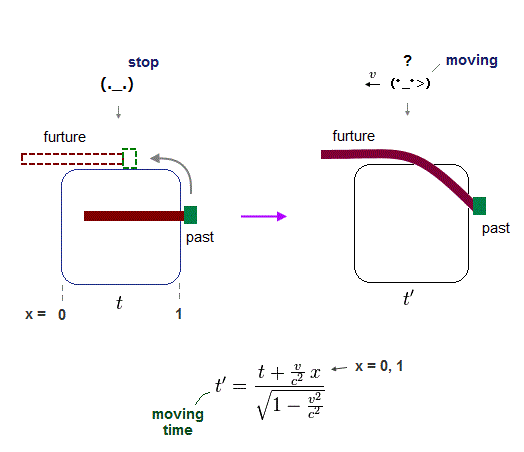Einstein special relaivity is unrealistic.
Clock time ticks differently depending observer.

Rigid rod moving upward is bent by moving observer !
Because clock time speed is changing in different positions and observers.

When the rod is moving upward and then horizontally, it is contorted unrealistically, though the rod is rigid !

So Einstein relativity is wrong.

## Einstein can change "future" of the rod ?

### [ "Block" without touching the rod can change the rod shape ! ]

(Fig.49)  "Block" changes the rod "future" direction.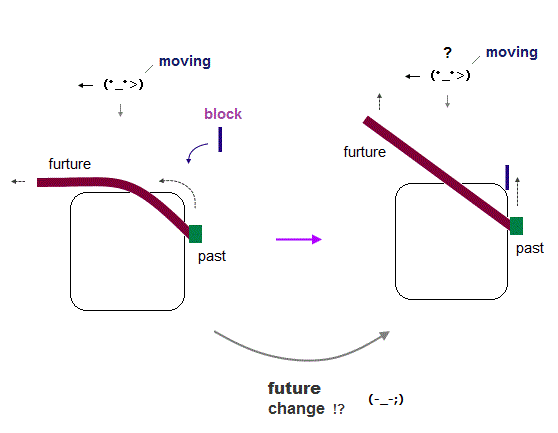The problem is that the only right part of the rod has NOT arrived at the turning point.  So this rod doesn't know whether there is some obstacle in the turning point.

If we insert some "block" in the turning point before the rod (= right part ) has arrived there, the whole rod cannot turn to the left, because the rod is rigid.

This means, the instant we insert a block, even the left horizontal part of the rod turns upward, though the block doesn't touch the rod !

This is clearly a fatal paradox, so special relativity is wrong.

### [ Magnetic force contradicts Einstein relativity. ]

(Fig.50)  Neutral current → "Positive" by observer's movement !In fact Einstein relativity includes fatal paradox also in electromagnetic force.

Magnetic field B is generated around a neutral electric current, and there is a stationary positive charge and observer in above-left figure.

But when this observer starts to move to the right, he sees the stationary charge moving in the opposite, so this charge feels downward magnetic force from the current.

To cancel this downward magnetic force, the above right current has to change from neutral to positive-charge ( this p.2 ) !

As a result, this charge don't move downward, seen by both obervers.
But strange phenomena.

## Observer moves  →  a charge is pulled !

### [ A negative charge is attracted, when observer moves !? ]

(Fig.51)  ↓ Einstein relativity shows "fatal" paradox !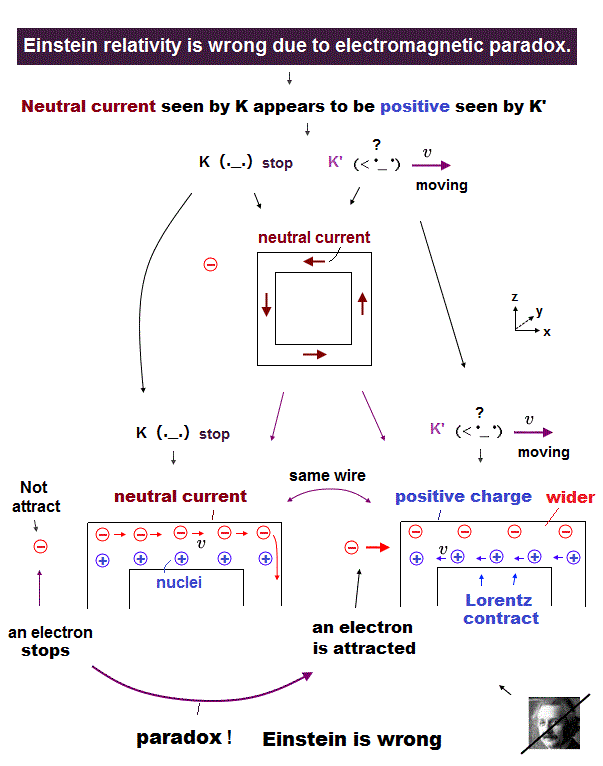This observer-dependent charged electric current causes paradox, when there is a stationary negative charge next to it.

When the observer starts to move and the current is positively charged, this negative charge is attracted to the current !

So a stationary observer in the above-left sees the negative charge is Not attracted to neutral current.

But a moving observer in the above-right sees the negative charge is attracted to the positively-charged current !

This is serious paradox, and Einstein special relativity is wrong.

Lorentz transformation of horizontal electric field E|| cannot handle the current charge ρ change ( this p.3 )

## Einstein mc2 is wrong.

### [ Mass increase depends on observer's movement ? ]

(Fig.52)  ↓ Mass (= energy ) change is "relative", NOT absolute value.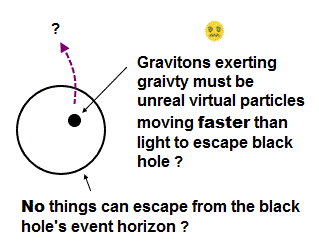Most textbooks claim that Einstein famous equation of E = mc2 contributes to nuclear power plant and atomic bomb.  But it's a total lie !

Because Einstein mass increase proportional to total energy has nothing to do with true energy.  It changes depending on observer !

Seen by stationary observer (= K ), the upper object is moving at v and increases its total kinetic energy and mass.

But seen by moving observer (= K' ), the upper object appears to stop, so its relativistic mass and energy do NOT increase.

In Einstein relativity, there is NO absolute true energy ( and mass ), because they change depending on observer's motion.

So you cannot utilize these "illusory" energy (= mass ) in actual atomic power generation and bombs.

Furthermore, Einstein mass relation causes serious paradox about force, so mc2 is wrong.

## Relativity contradicts de Broglie wave !

### [ de Broglie wavelength (= λ ) contradicts special relativity. ]

(Fig.53)  ↓ Electron's de Broglie wave vanishes !?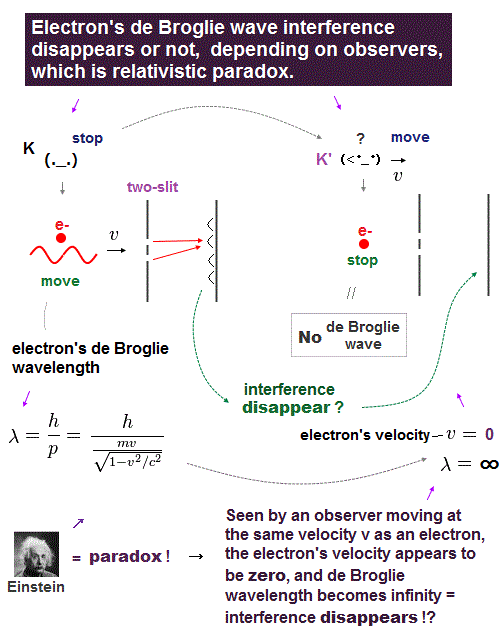The important point is Einstein relativity contradicts de Broglie wave relation.  So special relativity is false.

Electron's de Broglie wavelength was confirmed in various experiments.

In the above-left figure, an electron moving at v causes interference pattern at its de Broglie wavelength in two-slit experiment.

But seen by a moving observer at the same speed, this electron stops and generates No de Broglie wave, so No interference pattern is detected !

This is clearly a paradox of special relativity.

## How can we solve Einstein true paradoxes ?

### [ "Real medium" is needed to avoid serious paradoxes. ]

(Fig.54)  "Medium" solves every Einstein's problem !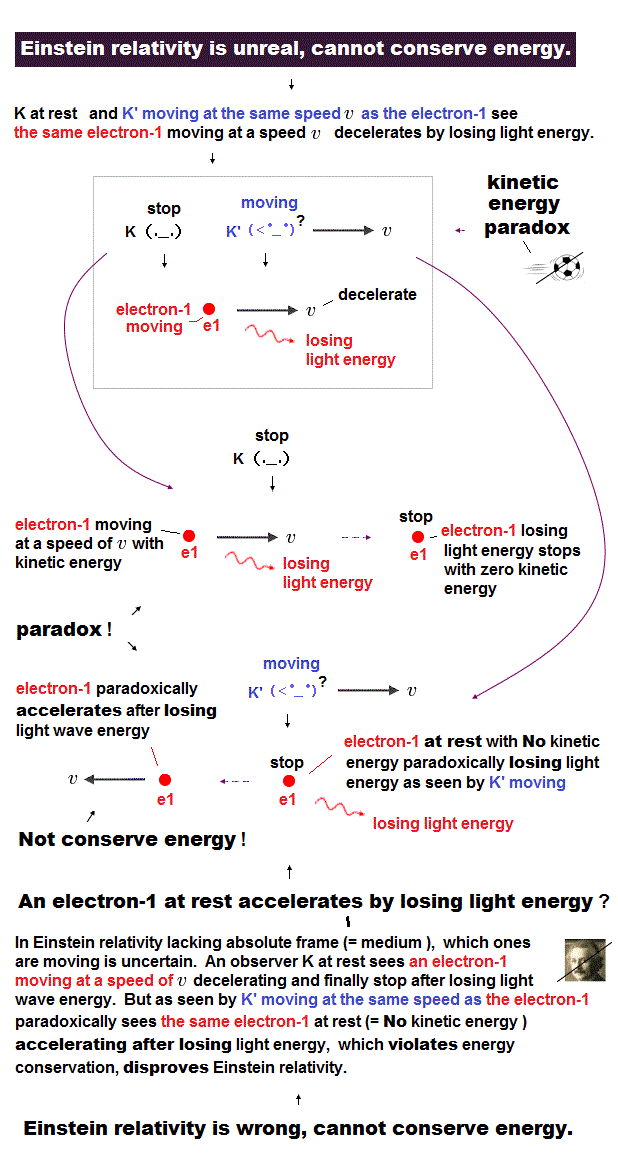Einstein special relativity causes serious paradoxes such as electromagnetic force, de Broglie wave, light refraction.

By introducing "real medium", we can solve every Einstein flaw.

When an electron is moving with respect to "medium", it generates de Broglie wave and magnetic field, which avoids paradoxes caused by observers.

Furthermore, "real medium" can explain dark matter, uniform cosmic microwave, gravity lens, increased mass, without unreal virtual photon.

## QED uses wrong math.

### [ Quantum electrodynamics (= QED ) uses deceptive method. ]

(Fig.55)  "Relativistic" QED disobeys Einstein !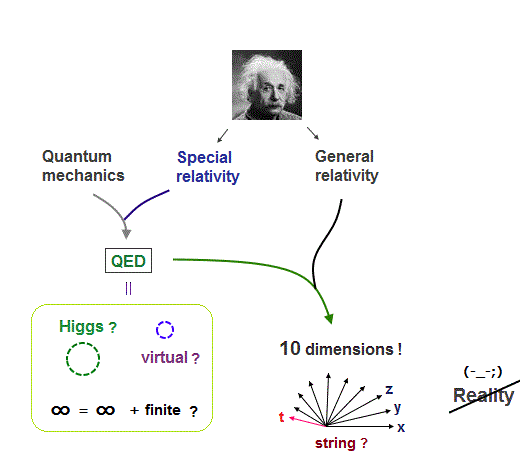Quantum electrodynamics (= QED ) is based on unreal virtual particles.

All calculation results by QED diverge to meaningless infinity.
They arficially subtract infinity from infinity to get finite value they like !

Due to this unreasonable math ( finite = ∞ - ∞ ), they can pretend to get accurate values.  So QED is Not accurate theory, but fraud method.

Many QED founders such as Dirac and Feynman criticized QED as wrong and"hocus-pocus".

QED has No influence on atomic behavior, but suddenly it claims infinite virtual particles appear to modify slightly small negligible value.  ← nonsense.

Methods of renormalization (= subtraction of infinity ) are artificially chosen to get conveninet values, so QED cannot predict any useful values, nonphysical.

## Antimatter is illusion.

### [ Positron emission = electron capture !? ]

(Fig.56)  ↓Positron emission is impossible.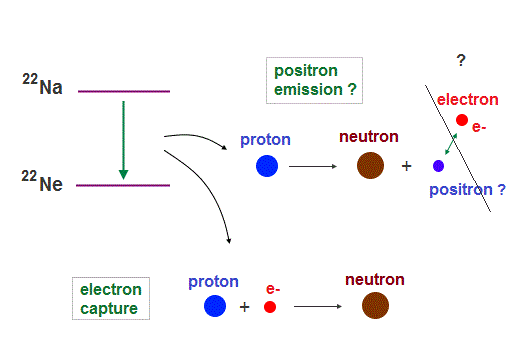PET is said to use positron (= antimatter ) emission.
But it is untrue, because this reaction is unrealistic.

First, positron itself cannot be detected, they just infuse some nuclide into patient and detect gamma rays.  That's all.

Positron emission is the same as electron capture, which don't need antimatter !

They assume that proton changes into heavier neutron and positron, which idea is ridiculous like perpetual machine.

There is no other way than to produce positive positron from proton, which is too firm to break !  Positron emission is unreal, replaced by electron cature.

Unstable antiparticle is illusion, just statistical artifact of other particles.

## Antimatter production = "momentum" is gone ?

### [ When the light changes into (anti)matter, its momentum is gone. ]

(Fig.57)  Light → positron + electron at rest ?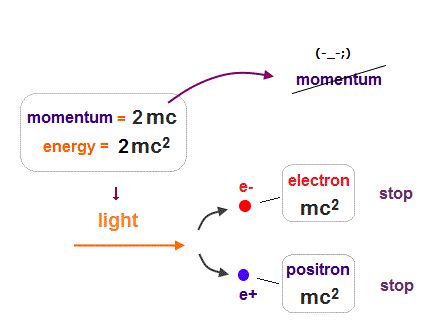It is said antimatter can be produced from high energy light (= γ ray ).  But we cannot generate it only from light !

They claim collision between accelerated electrons and nuclei is needed to generate antimatter.  Light involved in antimatter is vitual photon.

So "antimatter is produced from high enegy (real) γ ray" is misleading.
Furthermore, antimatter disobeys energy and momentum conservation !

When a light (= energy 2mc2 ) produces a pair of positron and electron at rest, the initial light momentum is gone, because the resultant pair is stationary.

The incident light always has momentum (= p ).  But after the light spends all its energy in producing a pair particles at rest, the initial momentum is missing !

So antiparticle production is based on unrealistic idea that only momentum ( without kinetic energy ) can transfer to other particle !

As a result, antiparticle doesn't exist.

## Black hole doesn't exist.

### [ Infinite time is needed to form black hole, so impossible. ]

(Fig.58)  Time stopping on black hole prevents its formation.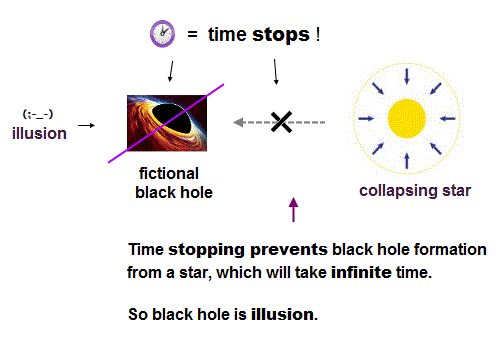Black hole doesn't exist.  Time stop around black hole prevents its formation.  Black hole contradicts special relativity.

Black hole has No evidence.  Even stars around black hole can Not be seen due to dense dusts.

They say the motion of S2 star (= closest to Milky way black hole ? ) might have proved massive black hole, but it is untrue.

This black hole mass is said to be 4 million Sun, so the cube root of this mass indicates that big star with 150 times Sun radius (= far shorter than the distance between S2 and black hole ) can explain this S2 motion.

So fantasy black hole is unneeded except for unreal extra dimension.

X-ray, they speculate, emitted from around black hole is based on doubtful assumption.

These high-energy lights are naturally thought from other sources such as nuclear fusion or something instead of fantasy black hole.

## Big Bang is fantasy.

### [ Universe expansion has No evidence. ]

(Fig.59)  Driving force to expand universe is dark energy ← NOT diluted ?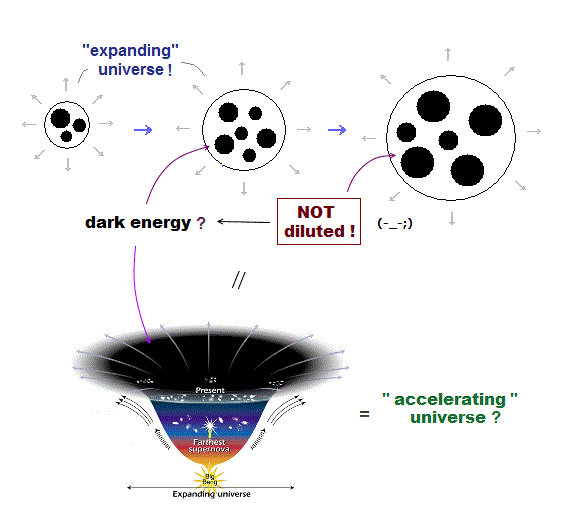Big Bang theory claims our universe is expanding, but earth and Sun are Not expanding !  This is strange.

If accelerating universe is true, its driving force = dark energy ? is Not diluted by expansion !

They say the most decisive proof of Big Bang is uniform cosmic microwave background.  But this is untrue.

They believe insane hypothesis that very weak microwave is intact (= Not affected by space dust ) even after it travels through space for 13.8 billion years !

It's natural to think uniform microwave proves the existence of uniform light medium in space, instead of fantasy Big Bang or multiverse.

Red-shifted lights from far away stars is due to loss of energy by being scattered by space dusts, rather than expanding universe.

All other gravitational wave, dark matter are unreal.

## Tunnel  is "classical", not quantum !

### [ Tunnel happens only when two atoms are "very close". ]

(Fig.60)  Tunnel current occurs only between very narrow gap ( ~ 1 Å )Scanning tunneling microscope is said to utilize tunnel effect where an electron can tunnel through "air", when some voltage bias is applied.

Only when the tip of microcope approaches the surface of material very closely (~ 1 Å ), the electric current flows between them.

They claim the "air" is an insulator which never allows an electron to pass.  So this microcope uses "magical" quantum tunnel effect ?

But it's natural that when two atoms get so close to each other, an electron can move into another atom from realistic (= classical ) viewpoint.

So tunnel has nothing to do with "occult" quantum mechanics.

## Quantum information is useless.

### [ Quantum key is just weak light.   = classical mechanics. ]

(Fig.61)  ↓ Weak light = eavesdropping is detected ?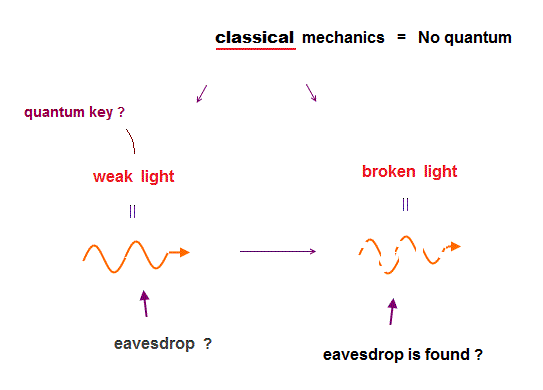"Quantum spooky action becoming practical" is fake news.

Entanglement (= spooky action ) can not send real information faster-than-light.

So physicists try to apply this useless concept to security in "cryptography".  But even in this communication security, quantum mechanics is useless.

Quantum key is just polarized classical light.  Weak light is fragile and easily destroyed by eavesdrop. ← Eavesdropping is detected by seeing destroyed light ?

But the weaker the light becomes, the more difficult it is for you to send light to distant place.  ← Impractical, forever.

## Entanglement is fiction.

### [ Physicists waste their time in useless fiction. ]

(Fig.62)  ↓ Entanglement is nonsense, Not science !Quantum entanglement (= spooky action ? ) can not send any real informations fastet-then-light.  So useless, waste of money.

In the above figure, the source emits a pair of lights with the same polarization (= vertical or horizontal is unknown ) in the opposite directions.

When you know the light A is vertically polarized, it means light B is vertically polarized, too.

They just call this state as "polarized lights A and B are entangled !"  ← Nonsense, Not science,  this entanglement cannot be used for anything.

Quantum teleportation sending nothing is useless, too.

Faster-than-light spooky action is illusion created by imaginary particle = photons, entangled photons can be explained by light classically.

## Realistic Bohr model helium.

### [ Avoiding destructive interference of two electrons' de Broglie waves ]

(Fig.63) Two de Broglie waves cross perpendicularly = stable.When two 1 × de Broglie wavelength orbits overlap on the same plane (= above-left figure ), opposite phases of electron's de Broglie cancel each other.

This is destructive interference between two electrons' de Broglie waves.

To avoid this cancellation, two electrons' orbits have to be perpendicular to each other.

This new Helium model gives surprisingly accurate experimental energy !

This calculation needs computer, which could Not be done in 1920s when they chose wrong theory = quantum mechanics.

## New Helium is stable.

(Fig.64) Old Bohr's circular helium = electrons are expelled.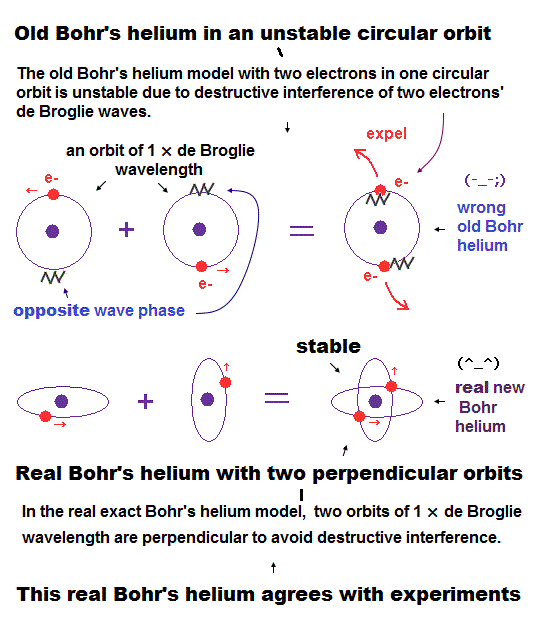In Old Bohr's circular helium, electrons are kicked out from orbits due to destructive interference between opposite de Broglie wave phases.

Two 1 × de Broglie wavelength electron orbits must cross each other perpendicularly to avoid cancellation.

No more electron orbit cannot enter this new Halium, so it can explain Pauli exclusion principle using de Broglie wave interference.

## New Bohr's helium satisfies 1 × de Broglie wavelength.

(Fig.65) Hydrogen and Helium atoms.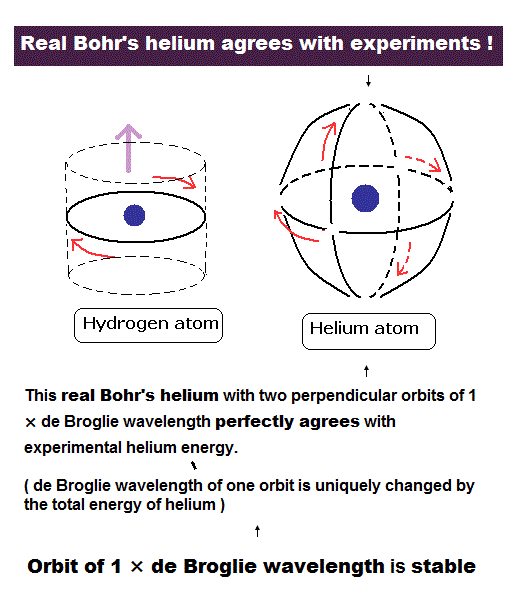These orbits are all just one de Broglie's wavelength.

In this new helium, the two symmetrical orbits crossing perpendicularly are wrapping the whole helium atom completely.

The Bohr model hydrogen which has only one orbit, can not wrap the direction of the magnetic moment completely.

This new helium model is just consistent with the fact of the strong stability and the closed shell property of helium.

In helium, the opposite ( same ) phases of two orbits move in the same ( opposite ) direction, which cancel de Broglie wave effect (= magnetic field ) at a distance, which is why helium magnet moment is zero.

But strictly speaking, Helium atom has small magnetic moment, so Helium is diamagnetic which repels (= creates the opposite magnentic field ) external magnetic field like classical Lenz's law.

Helium has magnetic moment = diamagnetic means quantum mechanical Helium with No angular momentum disagrees with actual Helium producing magnetic moment, so quantum mechanical model with unreal spin is wrong.

In free atoms like Helium, an electron's orbital radius tends to shrink in diamagnetism, which decreases total energy, hence helium becomes more stable (= Helium's two symmetrical electrons are thought to push each other in the opposite direction in the same external magnetic field and then prevent the whole Helium from flipping into paramagnetism. )

## Computing new Helium.

### [ Two orbits are perpendicular to each other, avoiding "destructive" interference. ]

(Fig.66) Two same-shaped orbital planes are perpendicular to each other.Next we calculate the new helium using simple computer program.
Fig.66 shows one quarter of the whole orbits.
We suppose electron 1 starts at ( r1, 0, 0 ), while electron 2 starts at ( -r1, 0, 0 ).

(Fig.67) The two electrons have moved one quarter of their orbitals.In Fig.67, after electrons move a quarter of their orbits, the electron 1 is crossing y axis perpendicularly, while electron 2 is crossing z axis.

## Computational methods.

Here we investigate how the electrons of the helium are moving by calculating the Coulomb force among the two electrons and the nucleus at short time intervals.

The computer programs of JAVA ( version 1.5.0 ), simple C languages and Python ( 2.7 ) to compute the electron orbit of the helium are shown in the link below.

### The program to calculate the electronic orbital of the helium

As shown in Fig.66 and Fig.67, the helium nucleus is at the origin.
The electron 1 initially at ( r1, 0, 0 ) moves one quarter of its orbit to ( 0, r2, 0 ), while the electron 2 initially at ( -r1, 0, 0 ) moves to ( 0, 0, r2 ).

As meter and second are rather large units for measurement of atomic behavior, here we use new convenient units

(Fig.68) New units of time and length.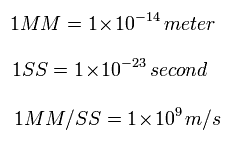From Fig.68, the accelaration is
(Fig.69)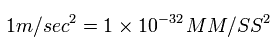If you copy and paste the above program source code into a text editor, you can easily compile and run this.

When you run this program ( for example, JAVA ) in command prompt, the following sentences are displayed on the screen.

(Fig.70)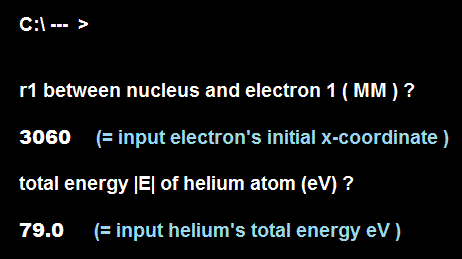First we input the initial x-coordinate r1 = r   (in MM) of electron 1 (see Fig.70 1 ), and press "enter" key.

In Fig.70, we input "3060", which means the initial x coordinate of electron 1 is 3060 MM = 3060 × 10-14 meter. The initial x coordinate of electron 2 becomes -3060 MM, automatically.

Next we input the absolute value of the total energy |E| (in eV) of helium.
In Fig.70, when we input "79.0", and press enter key, it means total energy of this helium is -79.0 eV.

(Fig.71) Initial states. "r" is initial x coordinate of electron 1.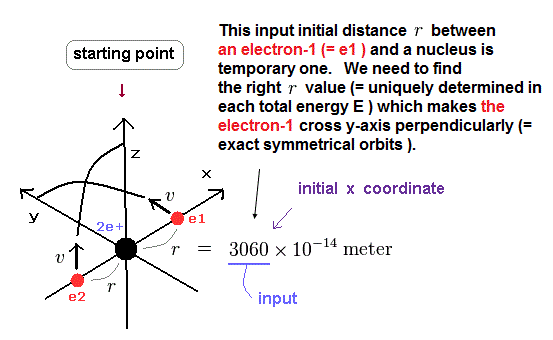From the inputted values, this program aturomatically calculates the initial velocity of the electron 1 ( = 2 ) in y ( z ) direction.

Total potential energy (= V ) of the initial state of Fig.71 becomes

(Fig.72) Initial total potential energy V.The first term of right side in Fig.72 is the potential energy between two electrons and 2e+ helium nucleus.

The second term is the repulsive potential energy between two electrons.

(Fig.73) Initial velocity "v".Total kinetic energy of two electrons is given by total energy (ex. -79.0 eV ) minus potential energy (= V ).

So from inputed values of Fig.70, we can get the initial velocity of each electron.
The initial velocity of electron 1 ( 2 ) is in y ( z ) direction.

(Fig.74) Change unit of velocity.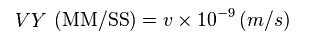Using the new unit of Fig.68, this program changes "m/s" into "MM/SS" in the initial velocity.
Because it is convenient when calculating each acceleration and de Broglie wave at intervals of 1 SS (= 10-23 seconds ).

## Computing Coulomb force at short time intervals.

(Fig.75) Positions of two electrons (= perpendicular and symmetric )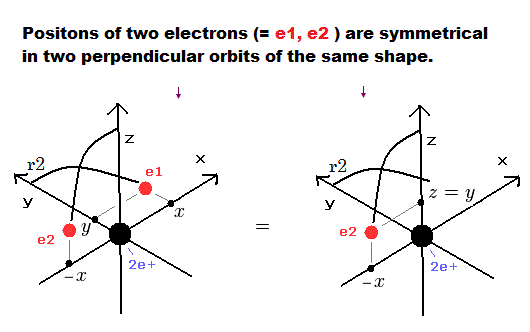At intervals of 1 SS, we compute the Coulomb force among the two electrons and the nucleus.

When the electron 1 is at ( x, y, 0 ), the electron 2 is at ( -x, 0, y ) due to their symmetric positions ( see Fig.66 and Fig.67 ).

So the x component of the acceleration ( m/sec2 ) of the electron 1 is,

(Fig.76) x component of the acceleration.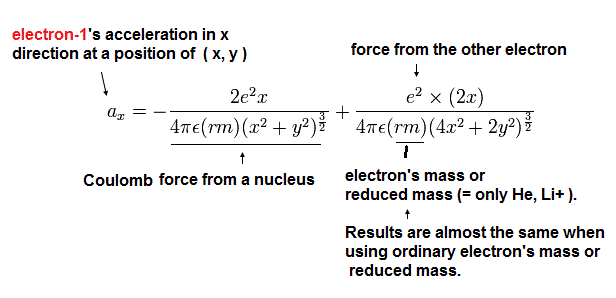where the first term is the Coulomb force between the nucleus and the electron 1, and the second term is the force between the two electrons.

(rm) is an electron's reduced mass.

(Fig.77) Distances among two electrons and nucleus.Due to symmetric positions of two electrons, when electron 1 is at ( x, y, 0 ), the electrons 2 is at ( -x, 0, z ), in which z = y.

As a result, the distance between electron 1 and nucleus is given by the first relation of Fig.77.
The second relation is the distance between two electrons.

## Calculation of acceleration in each direction.

Considering the helium nuclear mass (= alpha particle), we use here the reduced mass (= rm ) except when the center of mass is at the origin.

(Fig.78)  Reduced mass of one electron.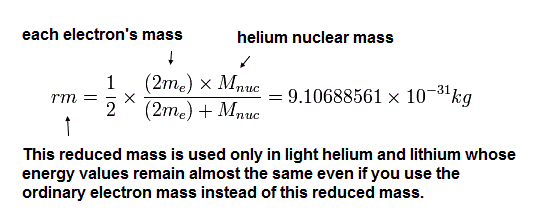In the same way, the y component of the acceleration (m/sec2) is,

(Fig.79) y component of the acceleration.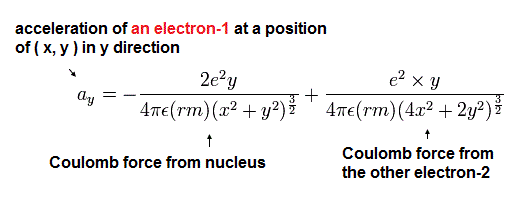Based on that calculation value, we change the velocity vector and the position of the electrons.

We suppose electron 1 moves only on the XY-plane, so the z component of the acceleration of the electron 1 is not considered.

If we consider all components of the Coulomb force against the electrons, the electron's motion becomes as shown in Fig. 64 upper.

But in this state, the two electrons are packed in one orbit of one de Broglie's wavelength where de Broglie wave oppsite phases (= ±ψ) are cancelled (= destructive interference ).

## Number of de Broglie waves contained in each short segment.

(Fig.80) De Broglie waves in each segment.We also calculate de Broglie wavelength of the electron from the velocity ( λ = h/mv ) at intervals of 1 SS.

The number of that wave ( λ in length ) contained in that short movement section is,

(Fig.81)  Number of de Broglie wavelength in the short segment.where (VX, VY) are the velocity of the electron 1 (in MM/SS ), the numerator is the movement distance (in meter) for 1 SS. the denominator is de Broglie's wavelength (in meter).

Here we use 1 MM = 10-14 meter.

Here, the estimated electron's orbit is divided into more than one million short segments for the calculation.

When the electron 1 has moved one quarter of its orbit and its x-coordinate is zero (Fig.82), this program checked the y-component of the electron 1 velocity (= last VY ).

Because "the last VY is zero" means two electrons are periodically moving around the nucleus in the same orbitals as shown in Fig.66 and Fig.67.

(Fig.82) Computing results   ( input: 79.00 eV, r1 = 3060 MM ).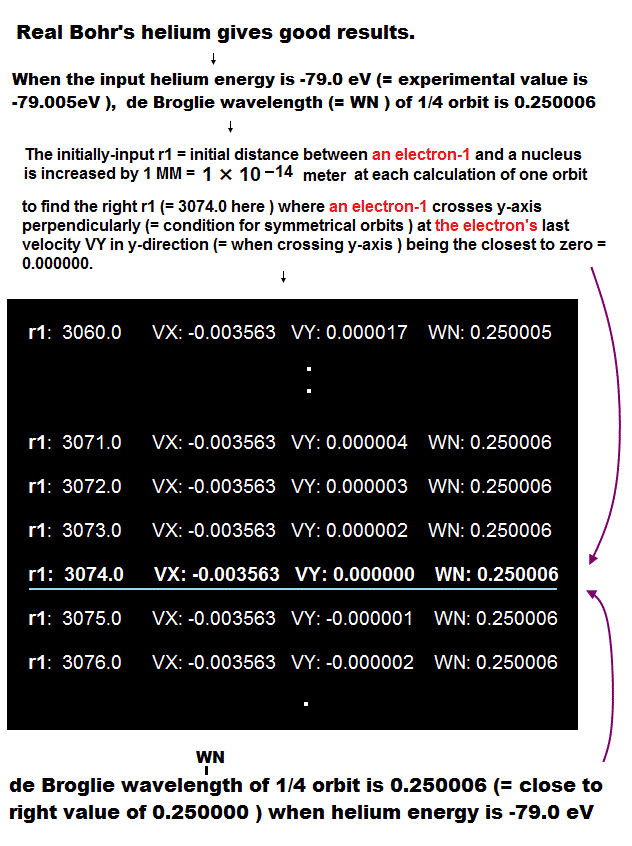After moving a quarter of the orbit, the program displays the above values on the screen.  The initial r1 automatically increases per each calculation of 1/4 orbit.

VX and VY are the last velocity of electron 1 ( MM/SS ).
preVY is the last y velocity 1ss before VY.

We pick up the values when this last VY is the closest to zero.
(mid)WN means the total number of de Broglie wavelength in one quarter of the orbit.

(Fig.83) When total energy is just -79.00 eV,   1/4 de Broglie wave is 0.250006.This program gives results when r1 increases from inputted value (ex. 3060 ) to r1+100 (= 3160 ).

As shown in Fig.82, when r1 is 3074 MM, last VY velocity of electron 1 becomes the smallest ( VY = 0.000000 ).

This means when r1 ( initial x coordinate ) = 3074 × 10-14 meter, these electron's orbits become just symmetric and electrons are stably moving in the same orbits.

In this case, the number of de Broglie wavelength contained in a quarter of its orbit becomes 0.250006.

So, one orbit is 0.250006 × 4 = 1.000024 de Broglie wavenlength. ( ← NOT 1.000000 )
As shown in Table 1, when inputted energy is -79.0037 eV, de Broglie wave becomes just 1.000000.

## Computing results agree with experimental value.

Table 1 shows the results in which the last VY is the closest to zero in different inputted total energies E.

This result shows when the total energy of new Bohr's helium is -79.0037 eV, each orbital length is just one de Broglie wavelength.

Table 1. Results.
E (eV) r1 (MM) WN WN x 4
-78.80 3082.0 0.250323 1.001292
-79.00 3074.0 0.250006 1.000024
-79.003 3074.0 0.250001 1.000004
-79.0037 3074.0 0.250000 1.000000
-79.005 3074.0 0.249998 0.999992
-79.01 3074.0 0.249990 0.999960
-79.20 3067.0 0.249690 0.998760

WN × 4 is the total number of de Broglie's wavelength contained in one round of the orbital.  This computed value is -79.0037 eV.

The experimental value of helium ground state energy is -79.005147 eV (= 1st + 2nd ionization energies, Nist, CRC ).

This result shows the relativistic correction (= resistance when closer to c ) to the energy = -79.005147 - (-79.0037 ) = -0.001447 eV.

The theoretical ground state energy value of the helium ion (He+) can be gotten from usual Bohr model or Schrodinger equation using the reduced mass.
This value is -54.41531 eV.

And the experimental value of He+ ground state energy is -54.41776 eV (Nist).
So the relativistic correction to the energy in He+ ion is -54.41776-(-54.41531) = -0.00245 eV.

The theoretical ground state energy value of the hydrogen atom (H) can be gotten from usual Bohr model or Schrodinger equation using the reduced mass, too.
This value is -13.5983 eV.

And the experimental value of H ground state energy is -13.59844 eV (Nist).
So the relativistic correction to the energy in hydrogen atom is -13.59844-(-13.5983) = -0.00014 eV.

## New Bohr helium agrees with experimental values.

The electron's velocity of the neutral helium atom is slower than helium ion, but faster than hydrogen atom.

So the relativistic correction in neutral helium atom should be between -0.00245 eV and -0.00014 eV.
The above calculation value of -0.001447 eV is just between them !

As a control program, we show the program of hydrogen-like atoms ( H and He+ ) using the same computing method as above. Try these, too.

Here we use the new unit ( 1 SS = 1 × 10-23 second ) and compute each value at the intervals of 1 SS.
If we change this definition of 1 SS, the calculation results of the total energy (E) in which the orbital length is just one de Broglie's wavelength change as follows,

Table 2.
1 SS = ? sec Result of E(eV)
1 × 10-22 -79.00540
1 × 10-23 -79.00370
1 × 10-24 -79.00355
1 × 10-25 -79.00350

This means that as the orbit becomes more smooth, the calculation values converge to -79.00350 eV.

The programs based on other 1 SS definition is as follows,
Sample JAVA program 1 SS = 1 × 10-25 sec, calculation takes much time.
Old sample JAVA program 1 SS = 1 × 10-22 sec--fast but the result and Eq.no are a little different

## New Bohr model holds good in all two and three atoms.

(Fig.84) Two-electron Atomic Model ( He, Li+, Be2+, B3+, C4+ ... )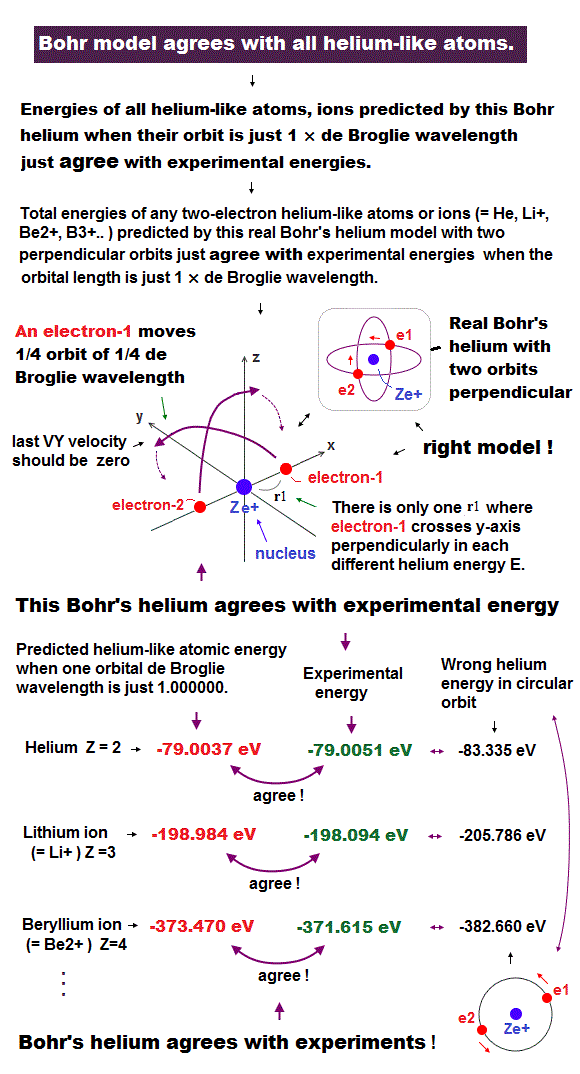Surprisingly, this new atomic structure of Bohr's helium is applicable to all other two and three electron atoms ( ions ).

JAVA program to compute two-electron atoms.

These program method is almost same as upper Helium, except you should input another information = atomic number (= Z ) after run this program.

See detailed computing method.

## Excellent results in two electron atoms !

(Table 3) Calculation results of two electron atoms (ions).
Atoms r1 (MM) WN x 4 Circular orbit Result (eV) ExperimentError (eV)
He 3074.0 1.000000 -83.335-79.0037 -79.0051 0.001
Li+ 1944.5 1.000000 -205.78-198.984 -198.093-0.89
Be2+ 1422.0 1.000000 -382.66-373.470 -371.615-1.85
B3+ 1121.0 1.000000 -613.96-602.32 -599.60-2.72
C4+ 925.0 1.000000 -899.67-885.6 -882.1-3.50
N5+ 788.0 1.000000 -1239.8-1223.3 -1219.1-4.20
O6+ 685.3 1.000000 -1634.38-1615.44 -1610.70-4.74
F7+ 607.3 1.000000 -2083.3-2062.0 -2057.0-5.00
Ne8+ 544.5 1.000000 -2586.7-2563.0 -2558.0-5.00

## Excellent results in three electron atoms !

Table 4 shows three electron atoms such as lithium.
See detailed computing method.

(Table 4) Calculation results of three electron atoms (ions).
Atoms r1 (MM) WN x 4 Result (eV) ExperimentError (eV)
Li 1949.0 1.000000 -203.033 -203.480 0.47
Be+ 1427.0 1.000000 -388.785 -389.826 1.04
B2+ 1125.0 1.000000 -635.965 -637.531 1.56
C3+ 928.0 1.000000 -944.46 -946.57 2.11
N4+ 790.5 1.000000 -1314.25 -1317.01 2.76
O5+ 688.0 1.000000 -1745.70 -1748.82 3.12
F6+ 609.4 1.000000 -2237.60 -2242.21 4.61
Ne7+ 546.0 1.000000 -2791.15 -2797.12 5.97

As shown here, we prove when two 1 × de Broglie wavelength orbits cross perpendicularly, they give surprisingly accurate energy results !

## Pauli exclusion principle by de Broglie wave.

### [ Two de Broglie opposite phases cross perpendicularly. ]

(Fig.85) ↓ Helium two electrons e1,e2 orbits are perpendicular.Here we explain why there are only two 1 × de Broglie wavelength orbits as shown in Pauli exclusion principle without unreal spin.

Helium has two 1 × de Broglie wavelength orbits.
Each orbit has only one electron because of 1 × wavelength.

1 × wavelength orbit consists of electron's phase (= thin line ) and its opposite phase (= thick line ).

Due to Coulomb repulsion between two electrons, each electron tend to be near the opposite phase of another electron.

To avoid destructive interference between two electrons, these two orbits have to be perpendicular to each other.

## Electrons do a U-turn at midpoint.

### [ Electron must do U-turn when opposite phase changes to harmless midpoint. ]

(Fig.86) ↓ Midpoint of de Broglie wave doesn't expel another electron.Each electron has to do a U-turn at some point keeping avoiding destructive interference from another electron's de Broglie wave.

In this Helium model, electrons do a U-turn when another de Broglie wave is midpoint between electron's same and opposite phases.

This midpoint is neutral phase, so it does't affect or destroy another electron's de Broglie wave.

So electrons can do a U-turn smoothly and safely, Not affected by aother de Broglie wave in this Helium model.

## Pauli exclusion principle.

### [ Why the maximum number of 1 × de Broglie wavelength orbits is two ? ]

(Fig.87) ↓ When there are four 1 × wavelength orbits, an electron is expelled.Here we explain why the maximum number of 1 × de Broglie wavelength orbits is two (= Pauli exclusion principle ).

When the total number of orbits is odd number ( ex. three = Not equilateral triangle ), it's unstable due to unbalanced, unsymmetric charge's distribution, so we think about four orbits.

When an atom contains four 1 × de Broglie wavelength orbits, each electron (ex. e3 ) is surrounded by opposite wave phases of other two orbits (= thick lines of e1, e2 ).

When an electron does a U-turn, it is expelled by destructive interference from other two opposite de Broglie wave phases.

So the two of four electrons in Beryllium cannot enter 1 × de Broglie wavelength orbits.

We can explain Pauli exclusion principle using de Broglie wave destructive interference without unreal spin !

## Neon uses the same principle as Helium.

### [ Neon consists of four 2 × de Broglie wavelength orbots. ]

(Fig.88) ↓ Elecrons cross the opposite phases perpendicularly like Helium.Like Helium, Neon can be explained by the same principle.
Neon has eight electrons.

We assume Neon consists of four 2 × de Broglie wavelength orbits, each orbit contains two electrons, so total is eight electrons.

As shown above figure, all electrons cross the opposite de Broglie wave phase of other electrons perpendicularly at vertices of hexahedron.

So Neon's electrons can avoid destructive interference of waves just like Helium.

## Neon electrons do a U-turn smoothly.

### [ 2 × de Broglie wavelength allows four orbits. ]

(Fig.89) ↓ Electrons do a U-turn at midpoints.When Helium comtains two 1 × de Broglie wavelength orbits, electrons can do a U-turn safely at neutral midpoints of other waves.

But when it contains four orbits, electrons are expelled , surrounded by other opposite wave phases, which is Pauli exclusion.

In case of 2 × de Broglie wavelength, four orbits enable electrons to move smoothly at midpoint, Not disturbed by destructive interference from other electron waves.

This is why 2 × de Broglie wavelength allows four orbits (= total electron number is eight. )

## The periodic motions of eight valence electrons in Neon.

### [ Neon 8 electrons in four 2 × de Broglie wavelength orbits. ]

(Fig.90)  Orbits of Neon cross each other "perpendicularly".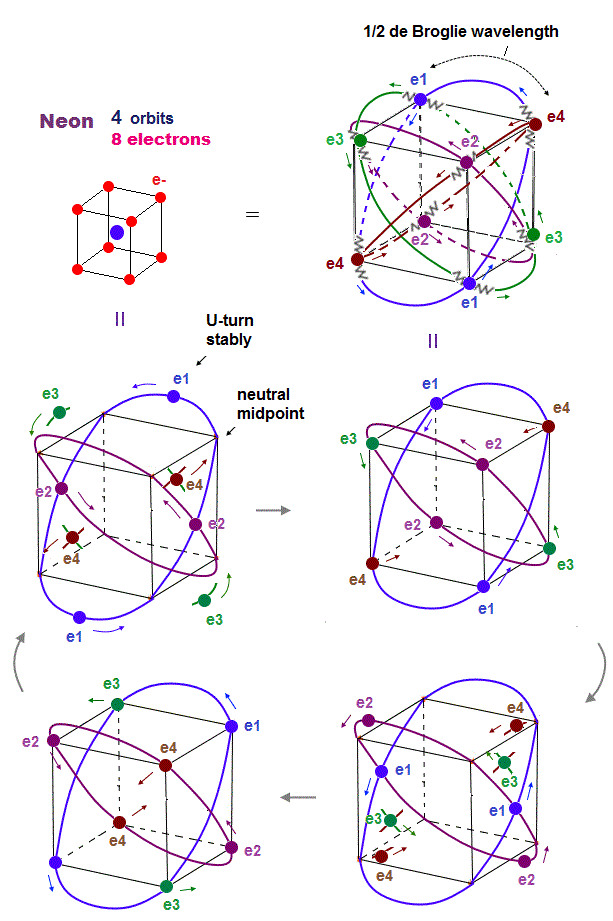As shown on this page, we can show the appropriate new Neon model, in which orbits can cross each other "perpendicularly".

"Perpendicular" crossing means they can avoid "destructive" interference.  Neon consists of four 2 × de Broglie wavelength orbits ( total 4 × 2 = 8 electrons )

## Generalized rules in atomc orbitals and de Broglie wavelength.

(Fig.91)  Maximum orbits = midpoint lines + 2 (= two perpendicular orbits )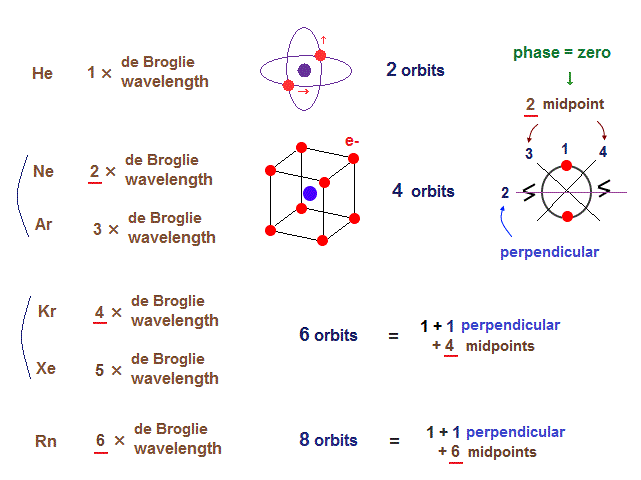In fact, all atoms use the same principle as Helium where two de Broglie waves cross perpendicularly.

Krypton consists of six 4 × de Broglie wavelength orbits.

If there are six 2 × de Broglie wavelength orbits, electrons are expelled surrounded by other opposite wave phases.

As a result, our new atomic model not only agrees with experimental results, but also explain Pauli principle without unreal spin !

## The maximum electrons' number.

### [ de Broglie wavelength determines valence electrons' number. ]

(Fig.92)  The opposite wave phases cross perpendicularly.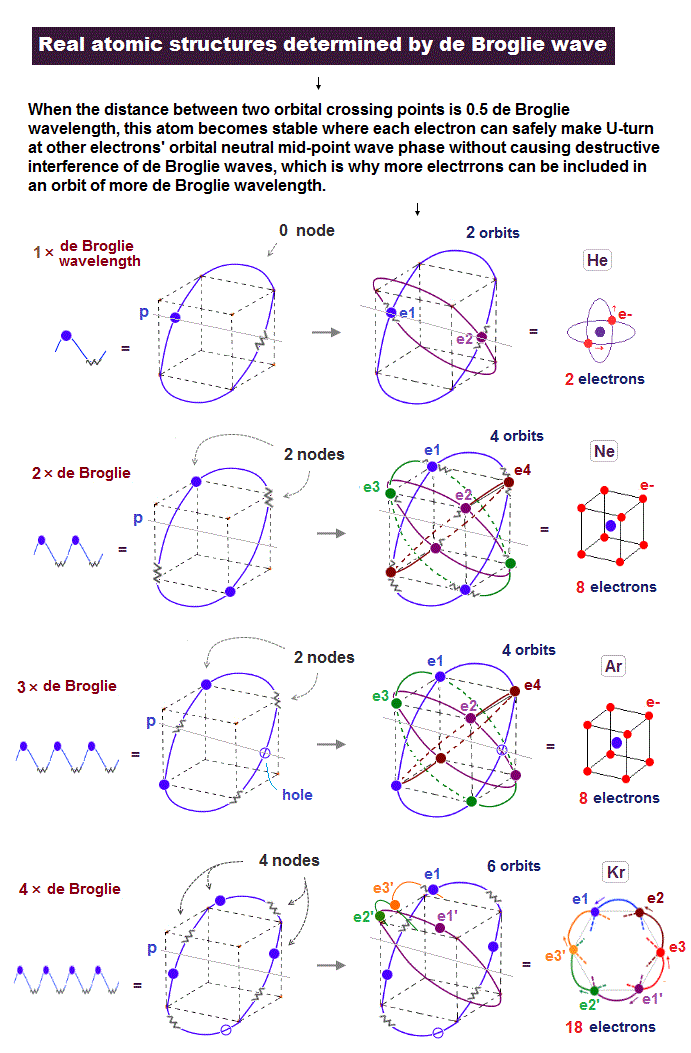Electrons spin is unrealistic and cannot explain the maximum valence electrons' number.

Using de Broglie wavelength, we can naturally explain Pauli principle and valence electrons' number without unreal spin.

Each orit crosses another orbit perpendicularly in the center line P (= 2 orbits ).

When the upper (or lower) part of each orbit inclues 2 nodes (= the opposite wave phases ), other 2 orbits need to cross the orbit so that all "pair" opposite wave phases cross perpendicularly.  ← The total is 2+2 = 4 orbits.

Neon (= Ne ) and Argon (= Ar ) have the same 2 nodes in the upper (or lower ) part of each orbit, so their total orbital numbers becomes the same 4 orbits, which can explain their same maximum valence electrons' number 8 (= 2 × 4 orbits ).

Krypton (= Kr ) is 4 × de Broglie wavelength, its orbit has 4 nodes in the upper (or lower ) part, so its total is 6 orbits (= 2 + 4 ), which can explain Kr valence electrons' number 18 (= 3 × 6 orbits )

## "Coulomb repulsions" must be considered.

### [ Argon has 8 valence electrons instead of 12 due to Coulomb repulsions. ]

(Fig.93)   If Ar has 12 valence electrons, Coulomb repulsion is Not uniform.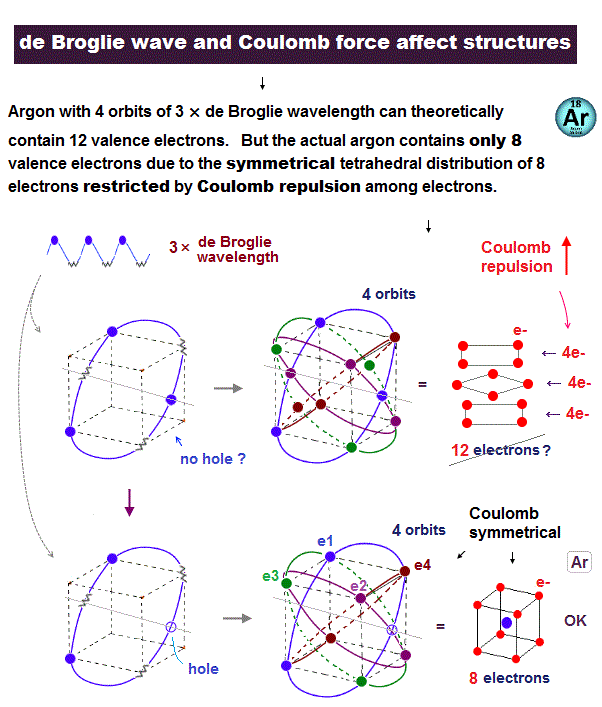Argon is 3 × de Broglie wavelength, and it has 4 orbits, so the total valence electrons should be 12 (= 3 × 4 orbits ) ?

Unfortunately, in this case, Coulomb repulsion among electrons is asymmetric (= Not uniform ) and stronger only in some parts, so unstable.

As shown above, if Ar has 12 electrons, it contains 3 layers of the same 4 electrons, which electrons' distribution is asymemtric.

For Coulomb repulsion to be uniformly (= symmetrically ) distributed around nucleus, the electrons' numbebrs of the upper and lower layers must be smaller than that of the middle layer (= the upper and lower layers have 2 electrons, and the middle layer has 4 electrons, the total is 8 electrons ).

When the total valence electron's number is 8 (= each orbit has 3 electrons + 1 hole ), the Ar valence elecrons are uniformly distributed in tetrahedron, so stable.

Coulomb repulsion must be considered also in Kr.

Classical orbit is far more useful and easier to handle in molecular calculation than unrel quantum mechanical wavefunction.

### [ Other pages. ]

♦ Valence electrons
♦ Bohr's Neon,   Carbon bonds,   Four-fundamental forces.
♦ de Broglie waves determine all atomic structures.
♦ Truth of electromagnetic waves.
♦ others   atomic size.

Japanese version2018/ 4/25 updated. Feel free to link to this site.